Скачать презентацию UNIVERSITY OF MASSACHUSETTS Dept of Electrical Computer

de86d0f166532899c784eb95bc273a6e.ppt

• Количество слайдов: 66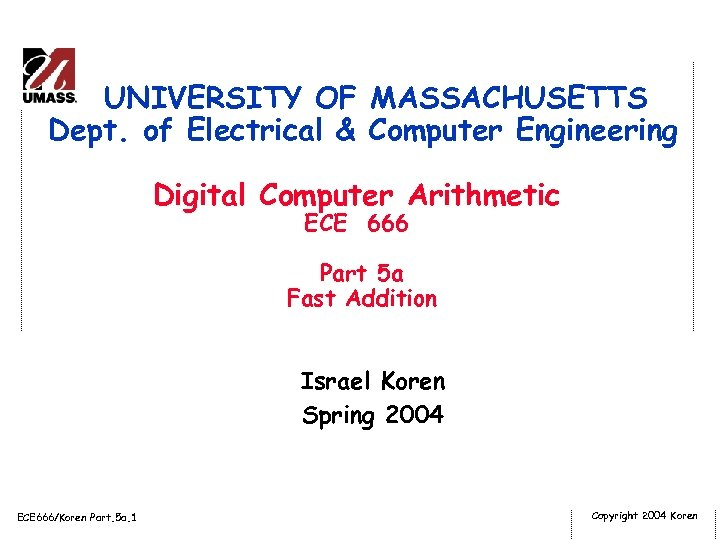UNIVERSITY OF MASSACHUSETTS Dept. of Electrical & Computer Engineering Digital Computer Arithmetic ECE 666 Part 5 a Fast Addition Israel Koren Spring 2004 ECE 666/Koren Part. 5 a. 1 Copyright 2004 Koren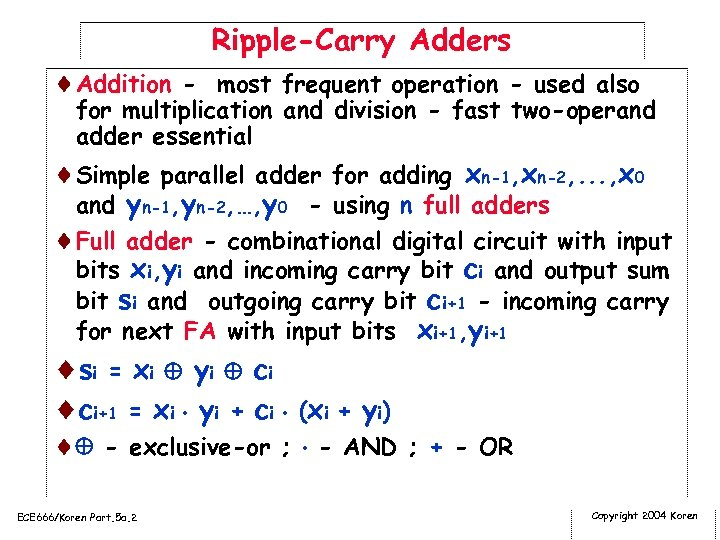Ripple-Carry Adders ¨Addition - most frequent operation - used also for multiplication and division - fast two-operand adder essential ¨Simple parallel adder for adding xn-1, xn-2, . . . , x 0 and yn-1, yn-2, …, y 0 - using n full adders ¨Full adder - combinational digital circuit with input bits xi, yi and incoming carry bit ci and output sum bit si and outgoing carry bit ci+1 - incoming carry for next FA with input bits xi+1, yi+1 ¨ s i = xi y i c i ¨ci+1 = xi yi + ci (xi + yi) ¨ - exclusive-or ; - AND ; + - OR ECE 666/Koren Part. 5 a. 2 Copyright 2004 Koren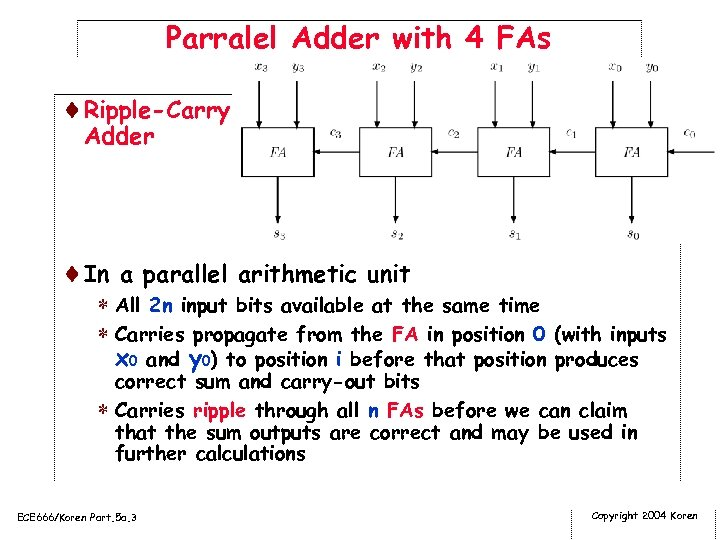Parralel Adder with 4 FAs ¨Ripple-Carry Adder ¨In a parallel arithmetic unit * All 2 n input bits available at the same time * Carries propagate from the FA in position 0 (with inputs x 0 and y 0) to position i before that position produces correct sum and carry-out bits * Carries ripple through all n FAs before we can claim that the sum outputs are correct and may be used in further calculations ECE 666/Koren Part. 5 a. 3 Copyright 2004 Koren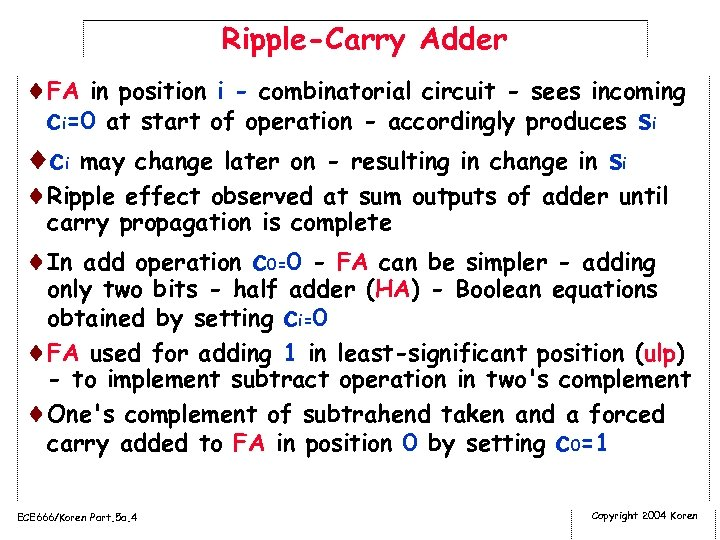Ripple-Carry Adder ¨FA in position i - combinatorial circuit - sees incoming ci=0 at start of operation - accordingly produces si ¨ci may change later on - resulting in change in si ¨Ripple effect observed at sum outputs of adder until carry propagation is complete ¨In add operation c 0=0 - FA can be simpler - adding only two bits - half adder (HA) - Boolean equations obtained by setting ci=0 ¨FA used for adding 1 in least-significant position (ulp) - to implement subtract operation in two's complement ¨One's complement of subtrahend taken and a forced carry added to FA in position 0 by setting c 0=1 ECE 666/Koren Part. 5 a. 4 Copyright 2004 Koren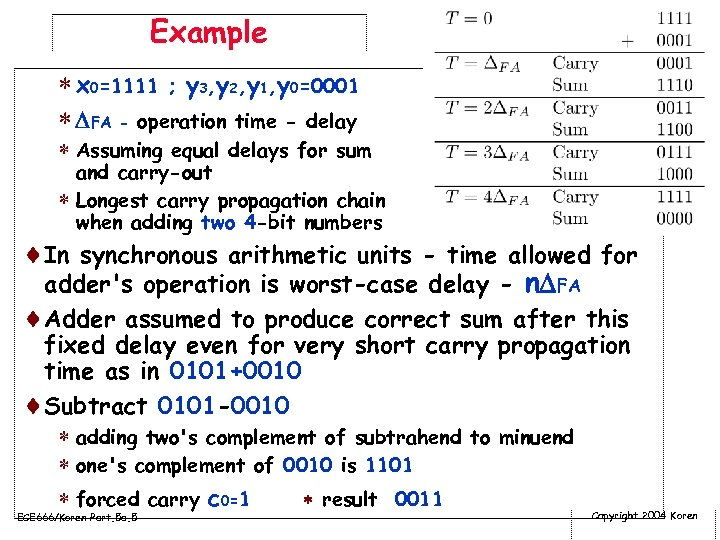Example * x 0=1111 ; y 3, y 2, y 1, y 0=0001 * FA - operation time - delay * Assuming equal delays for sum and carry-out * Longest carry propagation chain when adding two 4 -bit numbers ¨In synchronous arithmetic units - time allowed for adder's operation is worst-case delay - n FA ¨Adder assumed to produce correct sum after this fixed delay even for very short carry propagation time as in 0101+0010 ¨Subtract 0101 -0010 * adding two's complement of subtrahend to minuend * one's complement of 0010 is 1101 * forced carry c 0=1 result 0011 ECE 666/Koren Part. 5 a. 5 Copyright 2004 Koren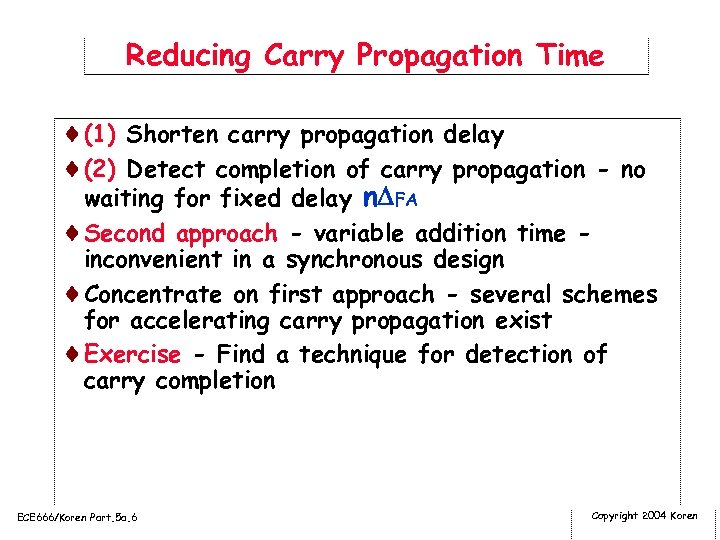Reducing Carry Propagation Time ¨(1) Shorten carry propagation delay ¨(2) Detect completion of carry propagation - no waiting for fixed delay n FA ¨Second approach - variable addition time - inconvenient in a synchronous design ¨Concentrate on first approach - several schemes for accelerating carry propagation exist ¨Exercise - Find a technique for detection of carry completion ECE 666/Koren Part. 5 a. 6 Copyright 2004 Koren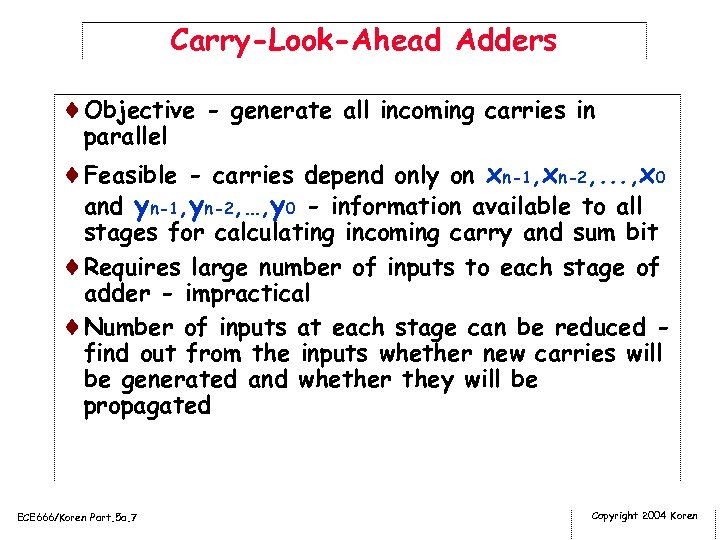Carry-Look-Ahead Adders ¨Objective - generate all incoming carries in parallel ¨Feasible - carries depend only on xn-1, xn-2, . . . , x 0 and yn-1, yn-2, …, y 0 - information available to all stages for calculating incoming carry and sum bit ¨Requires large number of inputs to each stage of adder - impractical ¨Number of inputs at each stage can be reduced find out from the inputs whether new carries will be generated and whether they will be propagated ECE 666/Koren Part. 5 a. 7 Copyright 2004 Koren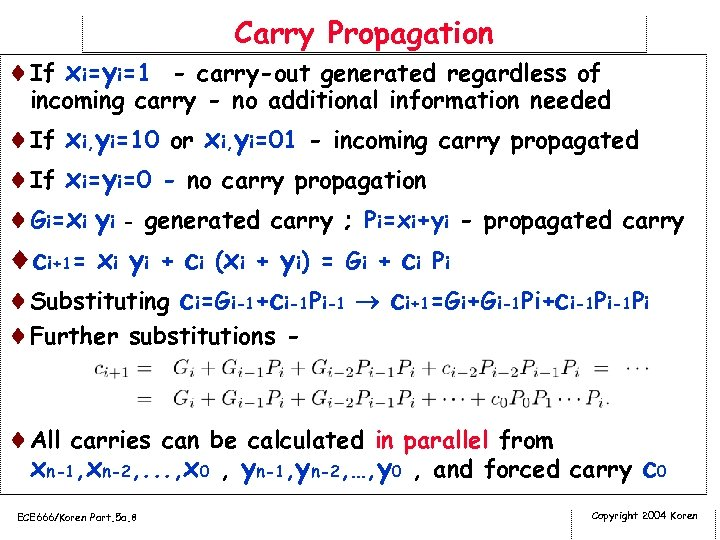Carry Propagation ¨If xi=yi=1 - carry-out generated regardless of incoming carry - no additional information needed ¨If xi, yi=10 or xi, yi=01 - incoming carry propagated ¨If xi=yi=0 - no carry propagation ¨Gi=xi yi - generated carry ; Pi=xi+yi - propagated carry ¨ci+1= xi yi + ci (xi + yi) = Gi + ci Pi ¨Substituting ci=Gi-1+ci-1 Pi-1 ci+1=Gi+Gi-1 Pi+ci-1 Pi ¨Further substitutions ¨All carries can be calculated in parallel from xn-1, xn-2, . . . , x 0 , yn-1, yn-2, …, y 0 , and forced carry c 0 ECE 666/Koren Part. 5 a. 8 Copyright 2004 Koren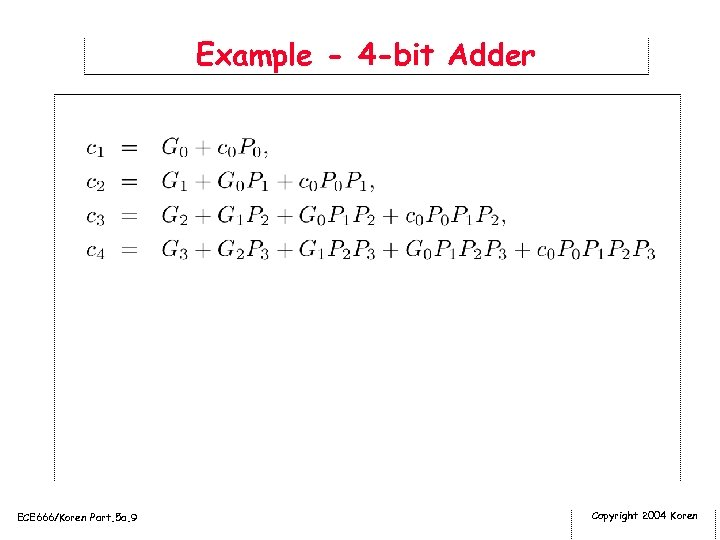Example - 4 -bit Adder ECE 666/Koren Part. 5 a. 9 Copyright 2004 KorenECE 666/Koren Part. 5 a. 10 Copyright 2004 Koren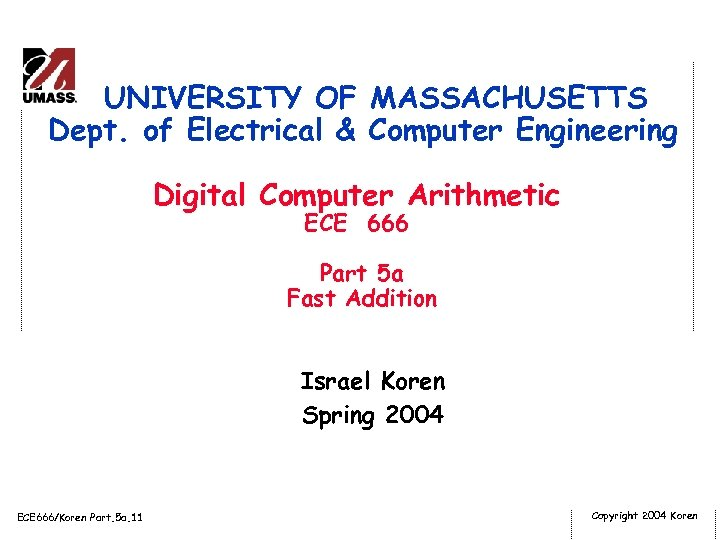UNIVERSITY OF MASSACHUSETTS Dept. of Electrical & Computer Engineering Digital Computer Arithmetic ECE 666 Part 5 a Fast Addition Israel Koren Spring 2004 ECE 666/Koren Part. 5 a. 11 Copyright 2004 Koren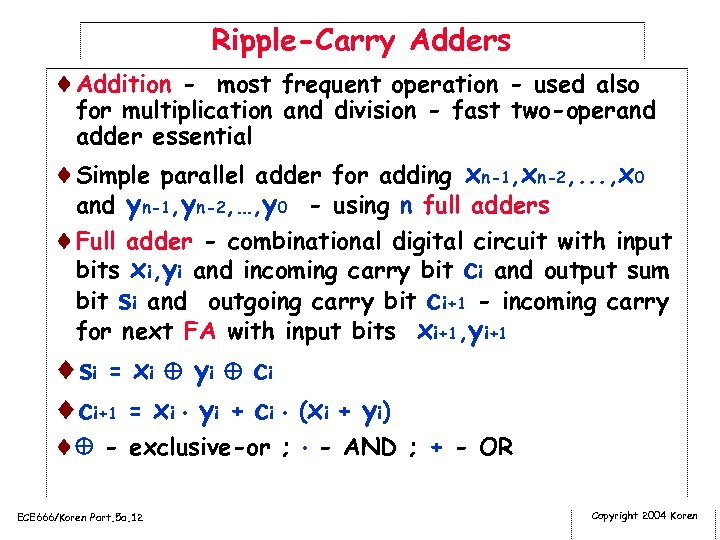Ripple-Carry Adders ¨Addition - most frequent operation - used also for multiplication and division - fast two-operand adder essential ¨Simple parallel adder for adding xn-1, xn-2, . . . , x 0 and yn-1, yn-2, …, y 0 - using n full adders ¨Full adder - combinational digital circuit with input bits xi, yi and incoming carry bit ci and output sum bit si and outgoing carry bit ci+1 - incoming carry for next FA with input bits xi+1, yi+1 ¨ s i = xi y i c i ¨ci+1 = xi yi + ci (xi + yi) ¨ - exclusive-or ; - AND ; + - OR ECE 666/Koren Part. 5 a. 12 Copyright 2004 Koren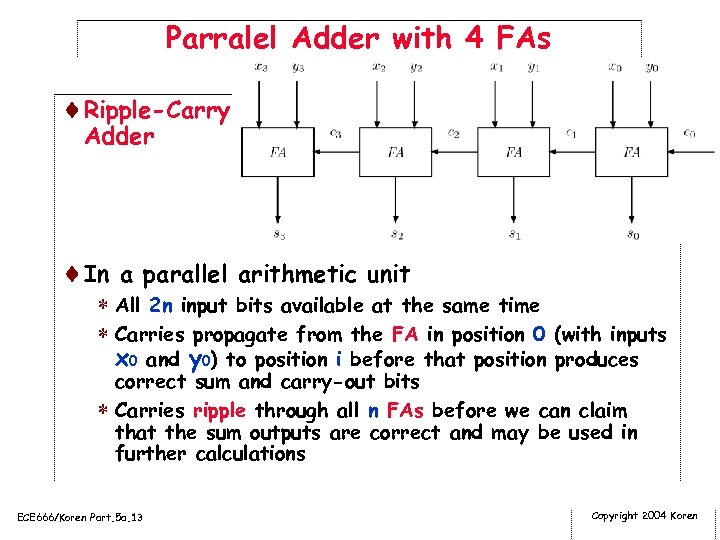Parralel Adder with 4 FAs ¨Ripple-Carry Adder ¨In a parallel arithmetic unit * All 2 n input bits available at the same time * Carries propagate from the FA in position 0 (with inputs x 0 and y 0) to position i before that position produces correct sum and carry-out bits * Carries ripple through all n FAs before we can claim that the sum outputs are correct and may be used in further calculations ECE 666/Koren Part. 5 a. 13 Copyright 2004 Koren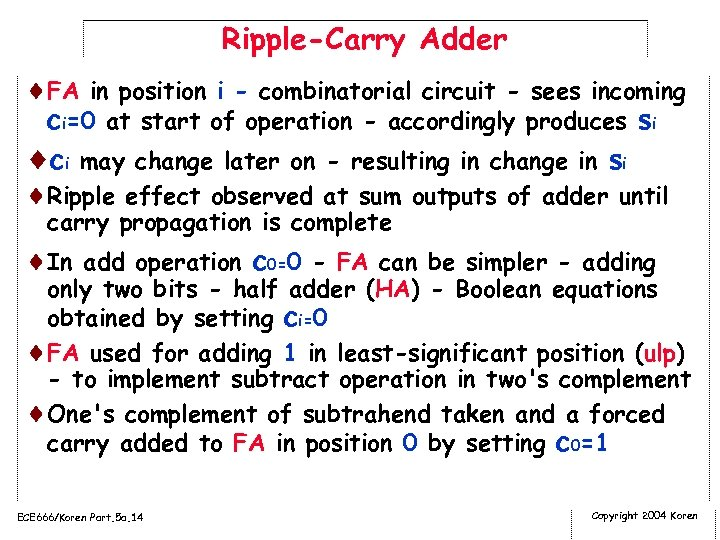Ripple-Carry Adder ¨FA in position i - combinatorial circuit - sees incoming ci=0 at start of operation - accordingly produces si ¨ci may change later on - resulting in change in si ¨Ripple effect observed at sum outputs of adder until carry propagation is complete ¨In add operation c 0=0 - FA can be simpler - adding only two bits - half adder (HA) - Boolean equations obtained by setting ci=0 ¨FA used for adding 1 in least-significant position (ulp) - to implement subtract operation in two's complement ¨One's complement of subtrahend taken and a forced carry added to FA in position 0 by setting c 0=1 ECE 666/Koren Part. 5 a. 14 Copyright 2004 Koren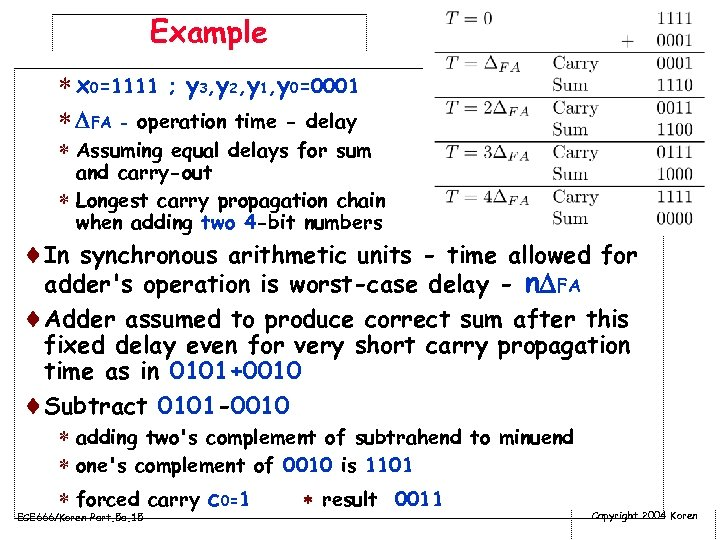Example * x 0=1111 ; y 3, y 2, y 1, y 0=0001 * FA - operation time - delay * Assuming equal delays for sum and carry-out * Longest carry propagation chain when adding two 4 -bit numbers ¨In synchronous arithmetic units - time allowed for adder's operation is worst-case delay - n FA ¨Adder assumed to produce correct sum after this fixed delay even for very short carry propagation time as in 0101+0010 ¨Subtract 0101 -0010 * adding two's complement of subtrahend to minuend * one's complement of 0010 is 1101 * forced carry c 0=1 result 0011 ECE 666/Koren Part. 5 a. 15 Copyright 2004 Koren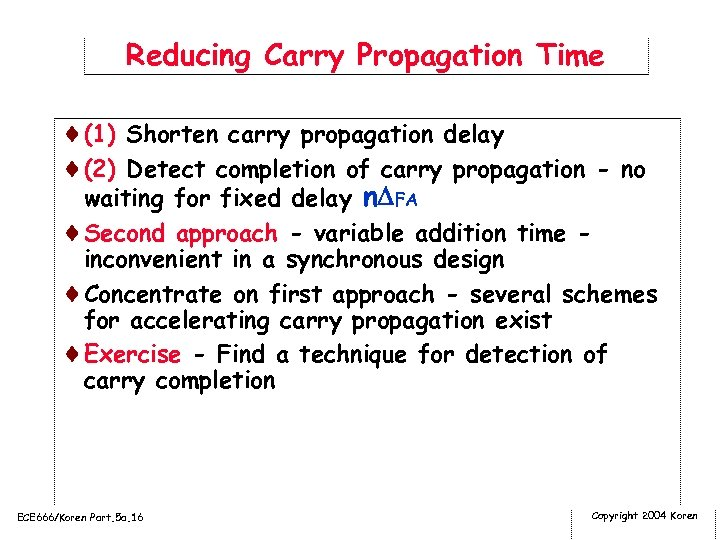Reducing Carry Propagation Time ¨(1) Shorten carry propagation delay ¨(2) Detect completion of carry propagation - no waiting for fixed delay n FA ¨Second approach - variable addition time - inconvenient in a synchronous design ¨Concentrate on first approach - several schemes for accelerating carry propagation exist ¨Exercise - Find a technique for detection of carry completion ECE 666/Koren Part. 5 a. 16 Copyright 2004 Koren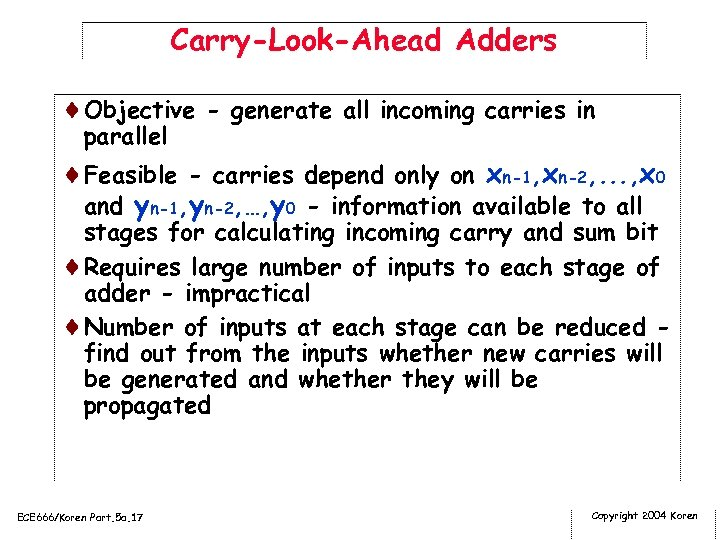Carry-Look-Ahead Adders ¨Objective - generate all incoming carries in parallel ¨Feasible - carries depend only on xn-1, xn-2, . . . , x 0 and yn-1, yn-2, …, y 0 - information available to all stages for calculating incoming carry and sum bit ¨Requires large number of inputs to each stage of adder - impractical ¨Number of inputs at each stage can be reduced find out from the inputs whether new carries will be generated and whether they will be propagated ECE 666/Koren Part. 5 a. 17 Copyright 2004 Koren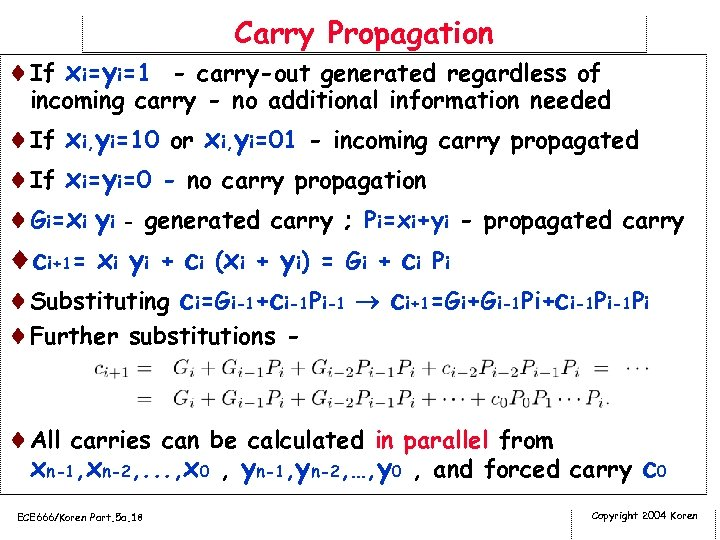Carry Propagation ¨If xi=yi=1 - carry-out generated regardless of incoming carry - no additional information needed ¨If xi, yi=10 or xi, yi=01 - incoming carry propagated ¨If xi=yi=0 - no carry propagation ¨Gi=xi yi - generated carry ; Pi=xi+yi - propagated carry ¨ci+1= xi yi + ci (xi + yi) = Gi + ci Pi ¨Substituting ci=Gi-1+ci-1 Pi-1 ci+1=Gi+Gi-1 Pi+ci-1 Pi ¨Further substitutions ¨All carries can be calculated in parallel from xn-1, xn-2, . . . , x 0 , yn-1, yn-2, …, y 0 , and forced carry c 0 ECE 666/Koren Part. 5 a. 18 Copyright 2004 Koren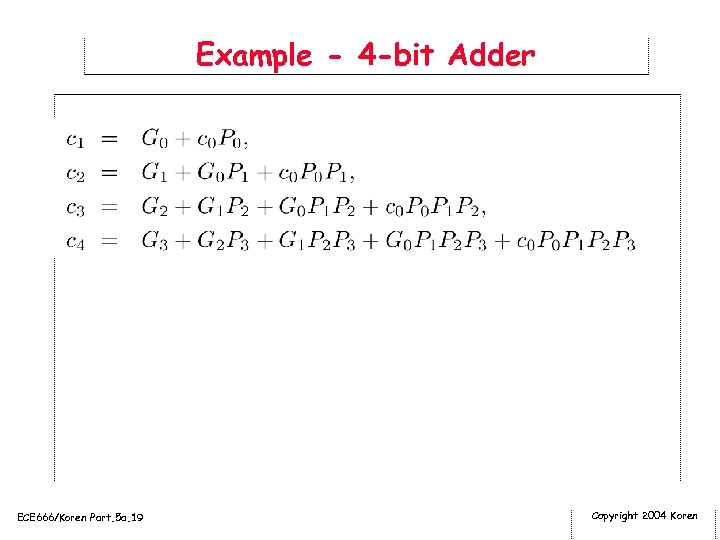Example - 4 -bit Adder ECE 666/Koren Part. 5 a. 19 Copyright 2004 Koren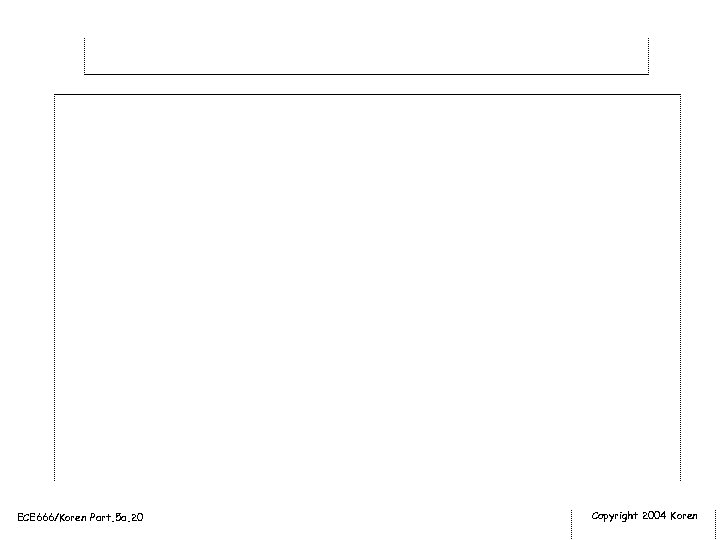ECE 666/Koren Part. 5 a. 20 Copyright 2004 Koren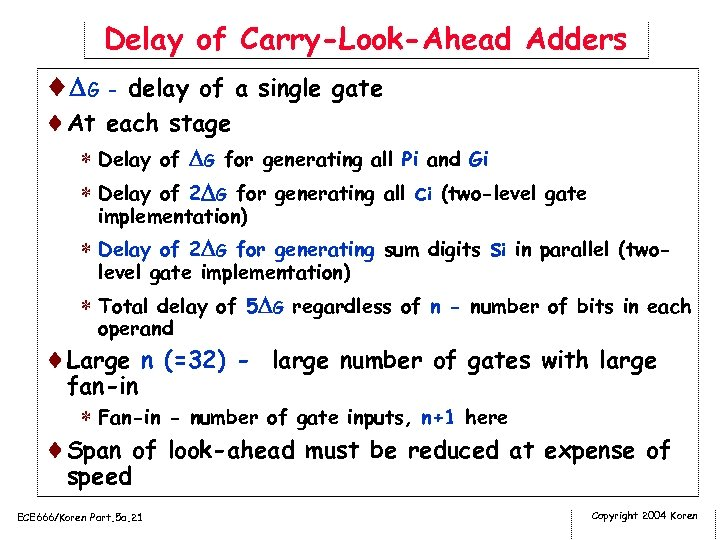Delay of Carry-Look-Ahead Adders ¨ G - delay of a single gate ¨At each stage * Delay of G for generating all Pi and Gi * Delay of 2 G for generating all ci (two-level gate implementation) * Delay of 2 G for generating sum digits si in parallel (twolevel gate implementation) * Total delay of 5 G regardless of n - number of bits in each operand ¨Large n (=32) - large number of gates with large fan-in * Fan-in - number of gate inputs, n+1 here ¨Span of look-ahead must be reduced at expense of speed ECE 666/Koren Part. 5 a. 21 Copyright 2004 Koren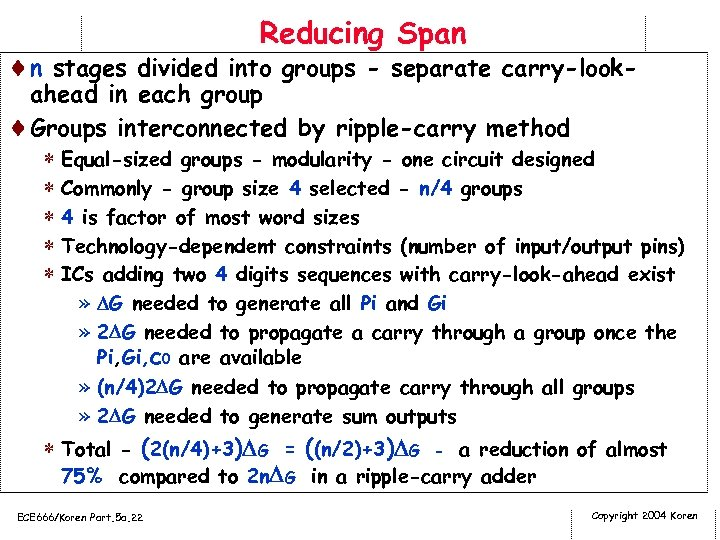Reducing Span ¨n stages divided into groups - separate carry-look- ahead in each group ¨Groups interconnected by ripple-carry method * Equal-sized groups - modularity - one circuit designed * Commonly - group size 4 selected - n/4 groups * 4 is factor of most word sizes * Technology-dependent constraints (number of input/output pins) * ICs adding two 4 digits sequences with carry-look-ahead exist » G needed to generate all Pi and Gi » 2 G needed to propagate a carry through a group once the Pi, Gi, c 0 are available » (n/4)2 G needed to propagate carry through all groups » 2 G needed to generate sum outputs * Total - (2(n/4)+3) G = ((n/2)+3) G - a reduction of almost 75% compared to 2 n G in a ripple-carry adder ECE 666/Koren Part. 5 a. 22 Copyright 2004 Koren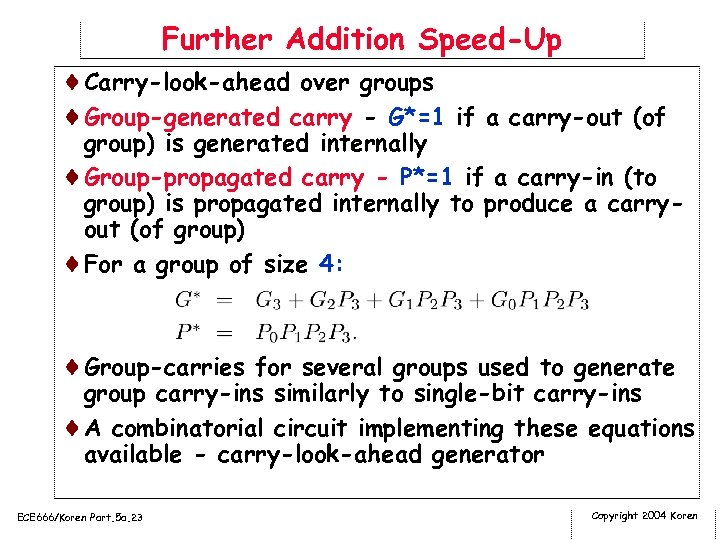Further Addition Speed-Up ¨Carry-look-ahead over groups ¨Group-generated carry - G*=1 if a carry-out (of group) is generated internally ¨Group-propagated carry - P*=1 if a carry-in (to group) is propagated internally to produce a carryout (of group) ¨For a group of size 4: ¨Group-carries for several groups used to generate group carry-ins similarly to single-bit carry-ins ¨A combinatorial circuit implementing these equations available - carry-look-ahead generator ECE 666/Koren Part. 5 a. 23 Copyright 2004 Koren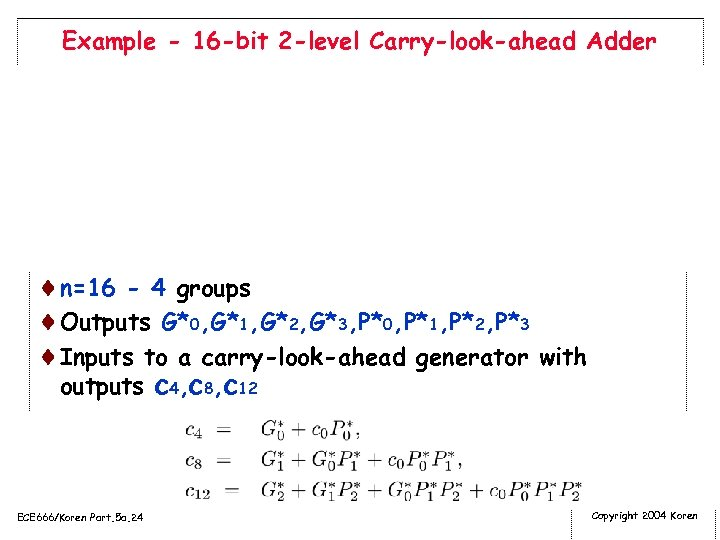Example - 16 -bit 2 -level Carry-look-ahead Adder ¨n=16 - 4 groups ¨Outputs G*0, G*1, G*2, G*3, P*0, P*1, P*2, P*3 ¨Inputs to a carry-look-ahead generator with outputs c 4, c 8, c 12 ECE 666/Koren Part. 5 a. 24 Copyright 2004 Koren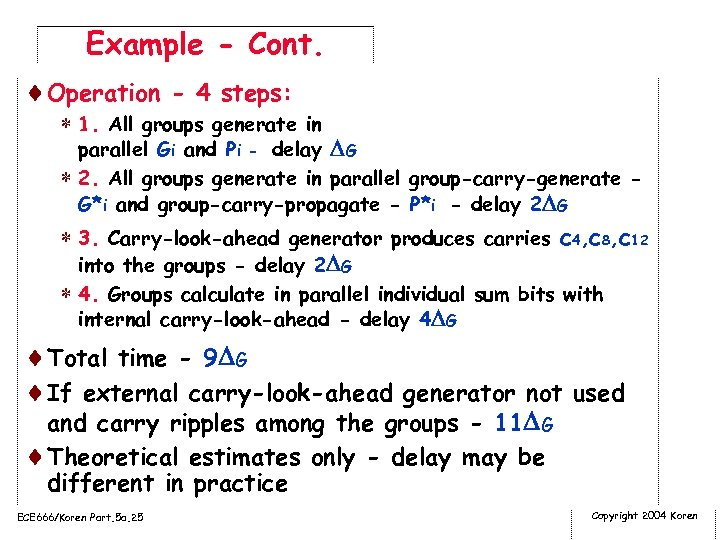Example - Cont. ¨Operation - 4 steps: * 1. All groups generate in parallel Gi and Pi - delay G * 2. All groups generate in parallel group-carry-generate G*i and group-carry-propagate - P*i - delay 2 G * 3. Carry-look-ahead generator produces carries c 4, c 8, c 12 into the groups - delay 2 G * 4. Groups calculate in parallel individual sum bits with internal carry-look-ahead - delay 4 G ¨Total time - 9 G ¨If external carry-look-ahead generator not used and carry ripples among the groups - 11 G ¨Theoretical estimates only - delay may be different in practice ECE 666/Koren Part. 5 a. 25 Copyright 2004 Koren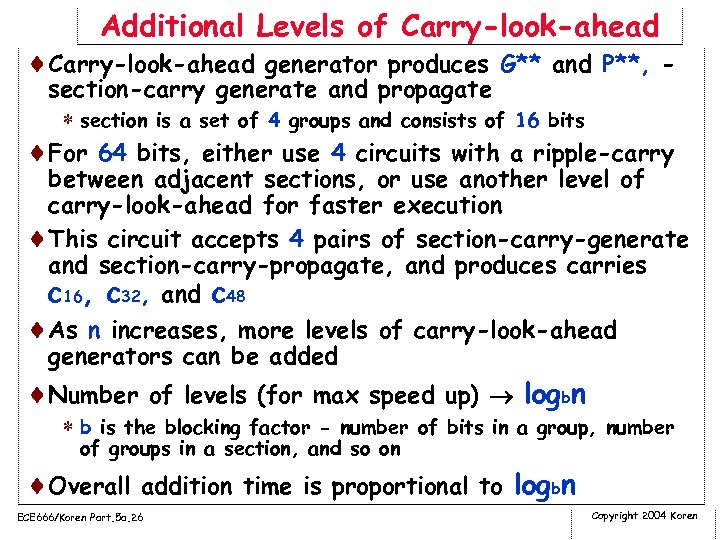Additional Levels of Carry-look-ahead ¨Carry-look-ahead generator produces G** and P**, - section-carry generate and propagate * section is a set of 4 groups and consists of 16 bits ¨For 64 bits, either use 4 circuits with a ripple-carry between adjacent sections, or use another level of carry-look-ahead for faster execution ¨This circuit accepts 4 pairs of section-carry-generate and section-carry-propagate, and produces carries c 16, c 32, and c 48 ¨As n increases, more levels of carry-look-ahead generators can be added ¨Number of levels (for max speed up) logbn * b is the blocking factor - number of bits in a group, number of groups in a section, and so on ¨Overall addition time is proportional to logbn ECE 666/Koren Part. 5 a. 26 Copyright 2004 Koren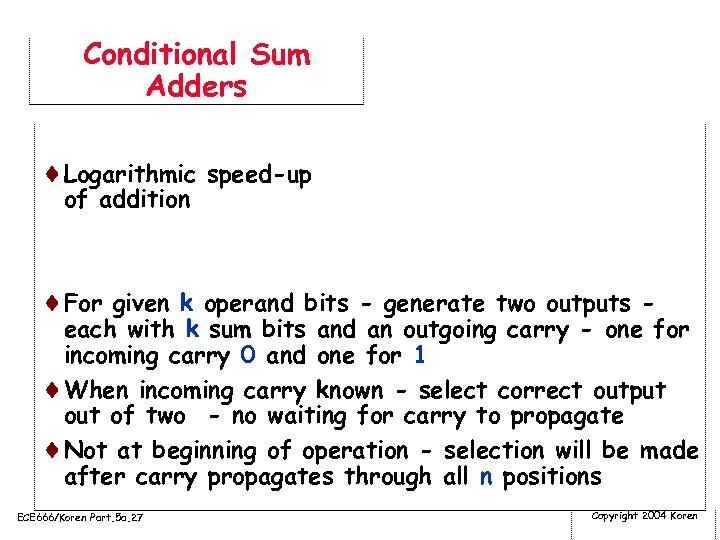Conditional Sum Adders ¨Logarithmic speed-up of addition ¨For given k operand bits - generate two outputs - each with k sum bits and an outgoing carry - one for incoming carry 0 and one for 1 ¨When incoming carry known - select correct output of two - no waiting for carry to propagate ¨Not at beginning of operation - selection will be made after carry propagates through all n positions ECE 666/Koren Part. 5 a. 27 Copyright 2004 Koren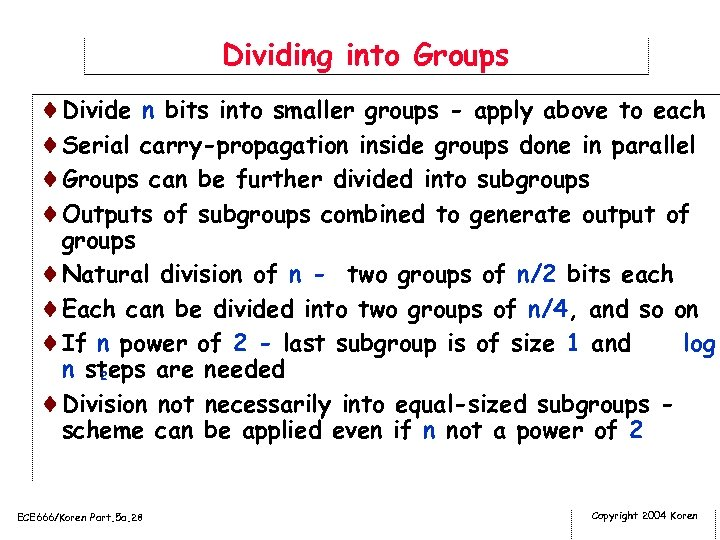Dividing into Groups ¨Divide n bits into smaller groups - apply above to each ¨Serial carry-propagation inside groups done in parallel ¨Groups can be further divided into subgroups ¨Outputs of subgroups combined to generate output of groups ¨Natural division of n - two groups of n/2 bits each ¨Each can be divided into two groups of n/4, and so on ¨If n power of 2 - last subgroup is of size 1 and log n steps are needed 2 ¨Division not necessarily into equal-sized subgroups scheme can be applied even if n not a power of 2 ECE 666/Koren Part. 5 a. 28 Copyright 2004 Koren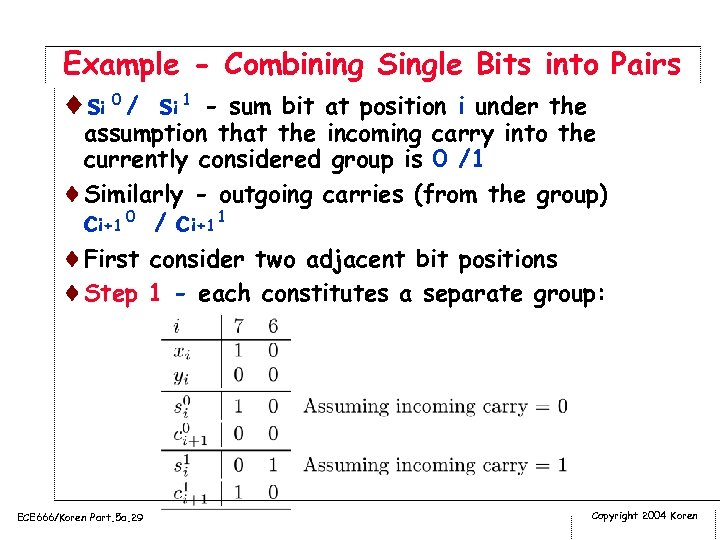Example - Combining Single Bits into Pairs ¨ si 0 / si 1 - sum bit at position i under the assumption that the incoming carry into the currently considered group is 0 /1 ¨Similarly - outgoing carries (from the group) ci+1 0 / ci+1 1 ¨First consider two adjacent bit positions ¨Step 1 - each constitutes a separate group: ECE 666/Koren Part. 5 a. 29 Copyright 2004 Koren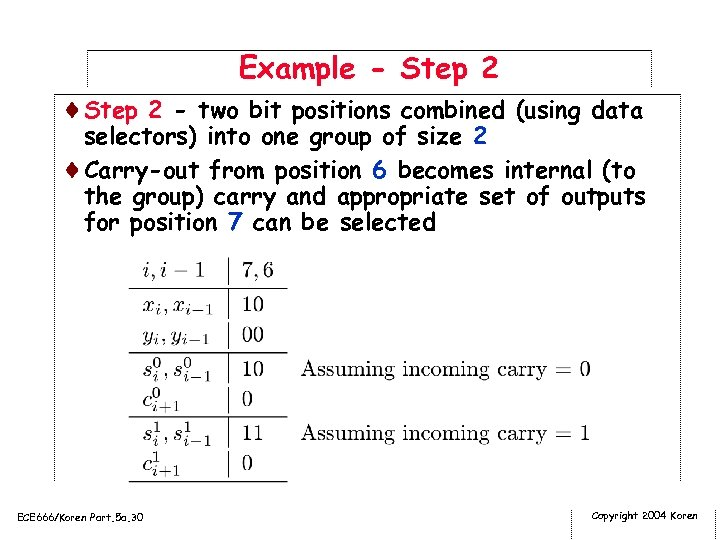Example - Step 2 ¨Step 2 - two bit positions combined (using data selectors) into one group of size 2 ¨Carry-out from position 6 becomes internal (to the group) carry and appropriate set of outputs for position 7 can be selected ECE 666/Koren Part. 5 a. 30 Copyright 2004 Koren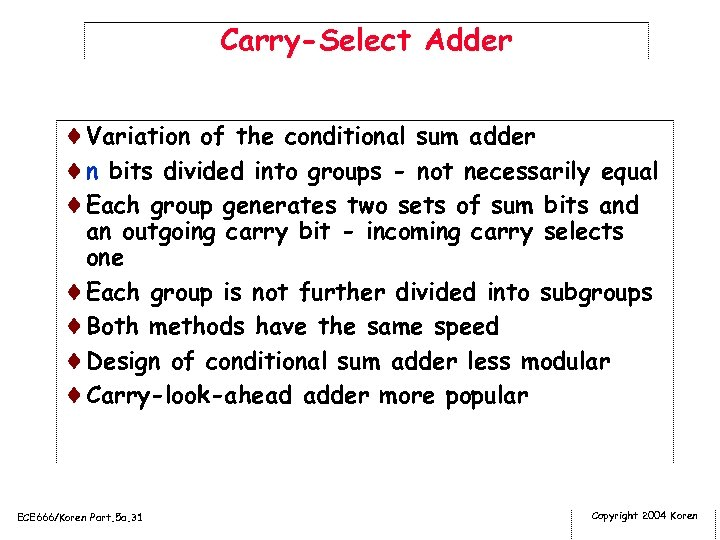Carry-Select Adder ¨Variation of the conditional sum adder ¨n bits divided into groups - not necessarily equal ¨Each group generates two sets of sum bits and an outgoing carry bit - incoming carry selects one ¨Each group is not further divided into subgroups ¨Both methods have the same speed ¨Design of conditional sum adder less modular ¨Carry-look-ahead adder more popular ECE 666/Koren Part. 5 a. 31 Copyright 2004 Koren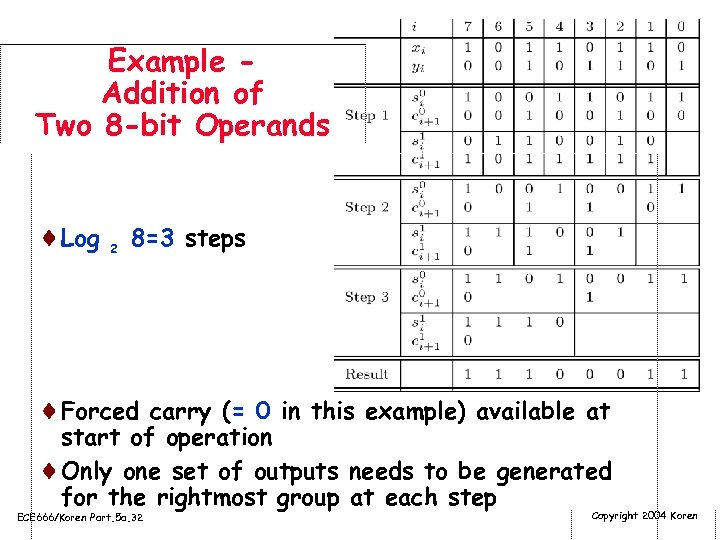Example Addition of Two 8 -bit Operands ¨Log 2 8=3 steps ¨Forced carry (= 0 in this example) available at start of operation ¨Only one set of outputs needs to be generated for the rightmost group at each step ECE 666/Koren Part. 5 a. 32 Copyright 2004 Koren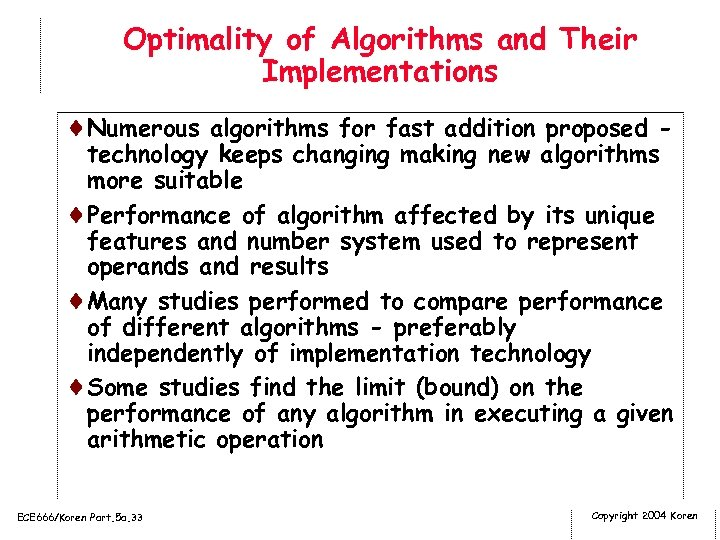Optimality of Algorithms and Their Implementations ¨Numerous algorithms for fast addition proposed - technology keeps changing making new algorithms more suitable ¨Performance of algorithm affected by its unique features and number system used to represent operands and results ¨Many studies performed to compare performance of different algorithms - preferably independently of implementation technology ¨Some studies find the limit (bound) on the performance of any algorithm in executing a given arithmetic operation ECE 666/Koren Part. 5 a. 33 Copyright 2004 Koren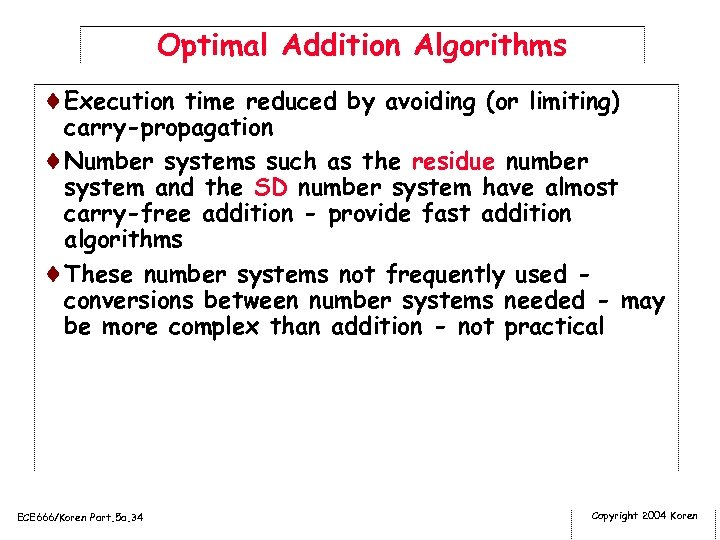Optimal Addition Algorithms ¨Execution time reduced by avoiding (or limiting) carry-propagation ¨Number systems such as the residue number system and the SD number system have almost carry-free addition - provide fast addition algorithms ¨These number systems not frequently used conversions between number systems needed - may be more complex than addition - not practical ECE 666/Koren Part. 5 a. 34 Copyright 2004 Koren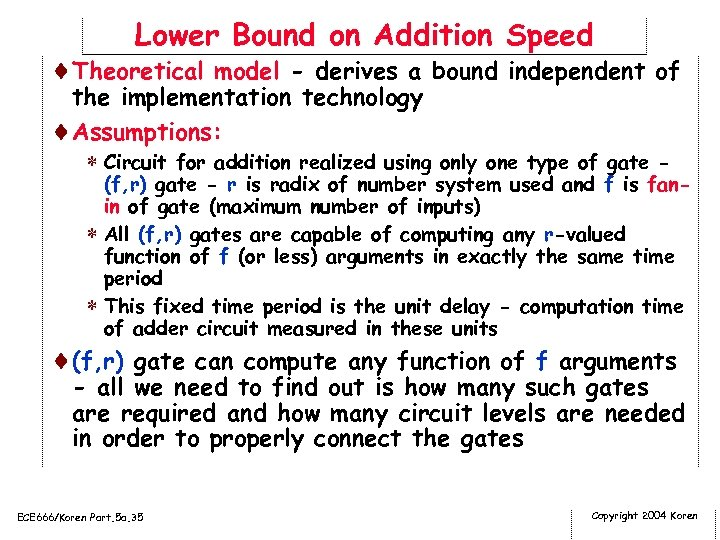Lower Bound on Addition Speed ¨Theoretical model - derives a bound independent of the implementation technology ¨Assumptions: * Circuit for addition realized using only one type of gate - (f, r) gate - r is radix of number system used and f is fanin of gate (maximum number of inputs) * All (f, r) gates are capable of computing any r-valued function of f (or less) arguments in exactly the same time period * This fixed time period is the unit delay - computation time of adder circuit measured in these units ¨(f, r) gate can compute any function of f arguments - all we need to find out is how many such gates are required and how many circuit levels are needed in order to properly connect the gates ECE 666/Koren Part. 5 a. 35 Copyright 2004 Koren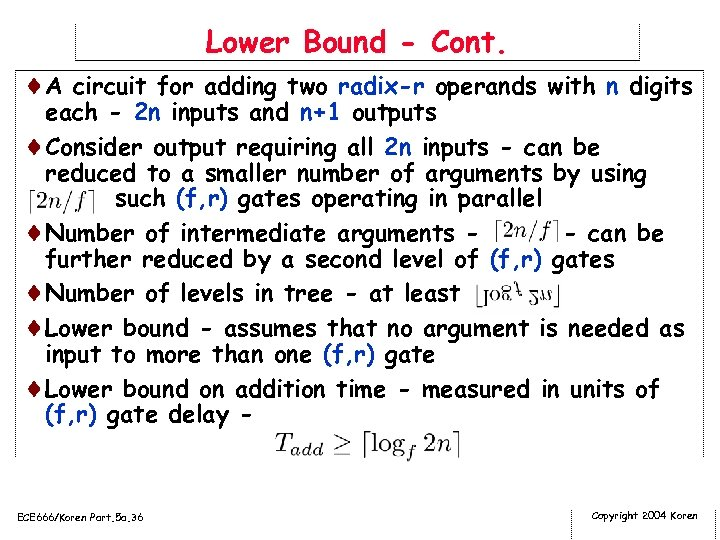Lower Bound - Cont. ¨A circuit for adding two radix-r operands with n digits each - 2 n inputs and n+1 outputs ¨Consider output requiring all 2 n inputs - can be reduced to a smaller number of arguments by using such (f, r) gates operating in parallel ¨Number of intermediate arguments - can be further reduced by a second level of (f, r) gates ¨Number of levels in tree - at least ¨Lower bound - assumes that no argument is needed as input to more than one (f, r) gate ¨Lower bound on addition time - measured in units of (f, r) gate delay - ECE 666/Koren Part. 5 a. 36 Copyright 2004 Koren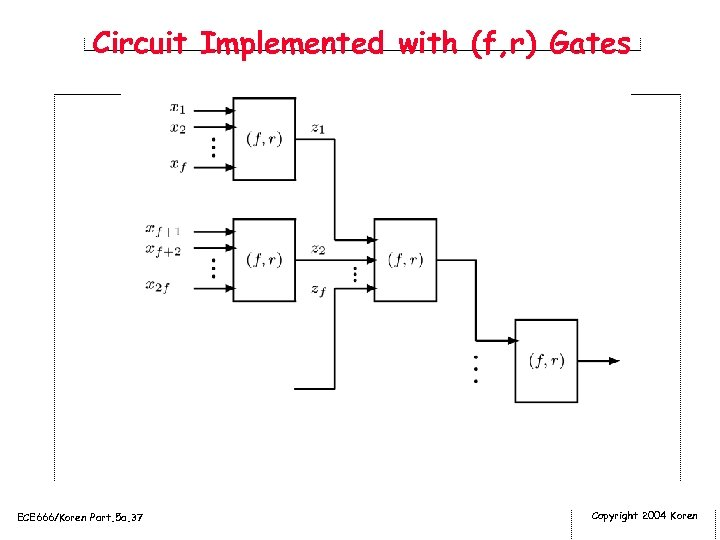Circuit Implemented with (f, r) Gates ECE 666/Koren Part. 5 a. 37 Copyright 2004 Koren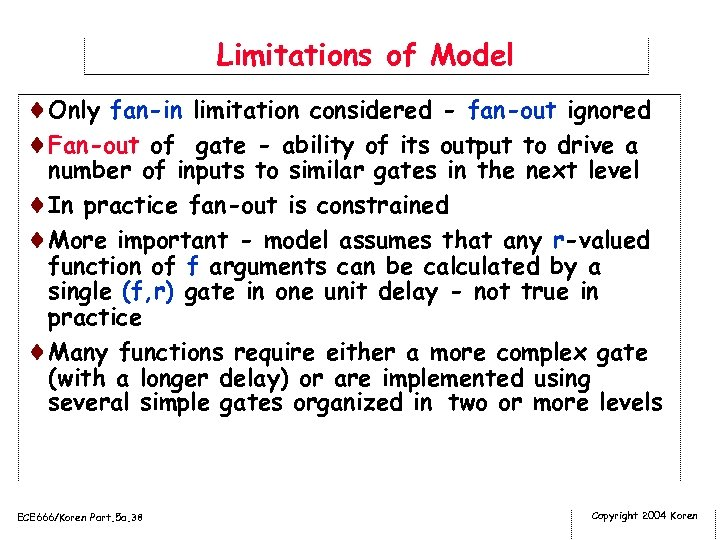Limitations of Model ¨Only fan-in limitation considered - fan-out ignored ¨Fan-out of gate - ability of its output to drive a number of inputs to similar gates in the next level ¨In practice fan-out is constrained ¨More important - model assumes that any r-valued function of f arguments can be calculated by a single (f, r) gate in one unit delay - not true in practice ¨Many functions require either a more complex gate (with a longer delay) or are implemented using several simple gates organized in two or more levels ECE 666/Koren Part. 5 a. 38 Copyright 2004 Koren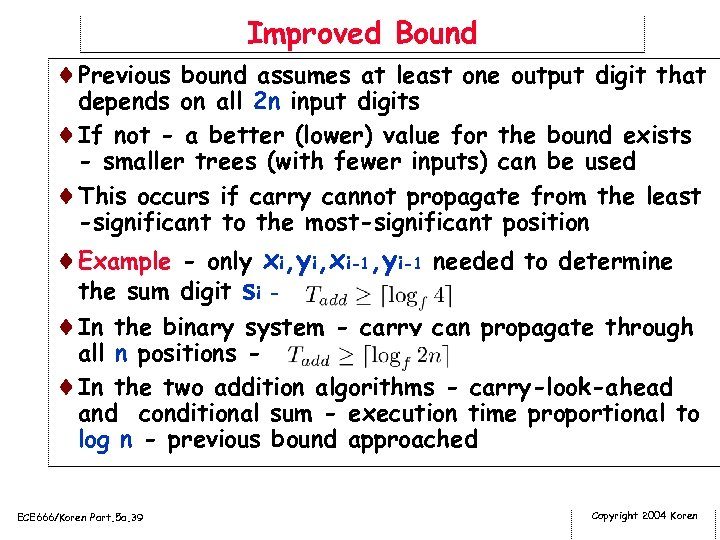Improved Bound ¨Previous bound assumes at least one output digit that depends on all 2 n input digits ¨If not - a better (lower) value for the bound exists - smaller trees (with fewer inputs) can be used ¨This occurs if carry cannot propagate from the least -significant to the most-significant position ¨Example - only xi, yi, xi-1, yi-1 needed to determine the sum digit si ¨In the binary system - carry can propagate through all n positions ¨In the two addition algorithms - carry-look-ahead and conditional sum - execution time proportional to log n - previous bound approached ECE 666/Koren Part. 5 a. 39 Copyright 2004 Koren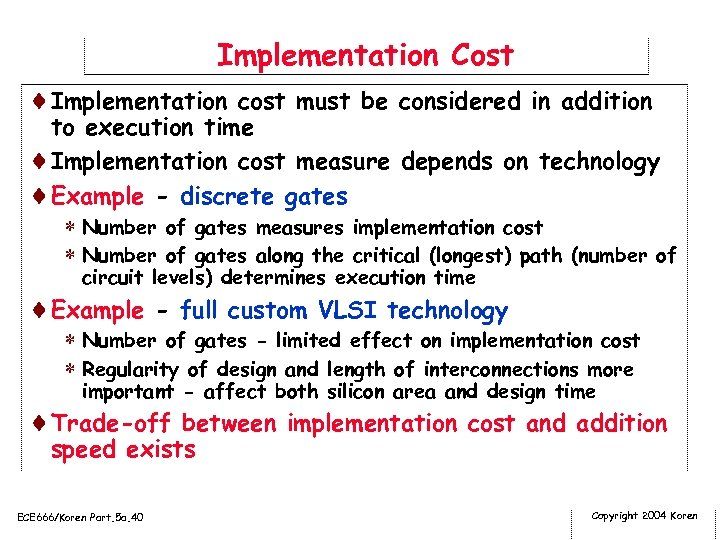Implementation Cost ¨Implementation cost must be considered in addition to execution time ¨Implementation cost measure depends on technology ¨Example - discrete gates * Number of gates measures implementation cost * Number of gates along the critical (longest) path (number of circuit levels) determines execution time ¨Example - full custom VLSI technology * Number of gates - limited effect on implementation cost * Regularity of design and length of interconnections more important - affect both silicon area and design time ¨Trade-off between implementation cost and addition speed exists ECE 666/Koren Part. 5 a. 40 Copyright 2004 Koren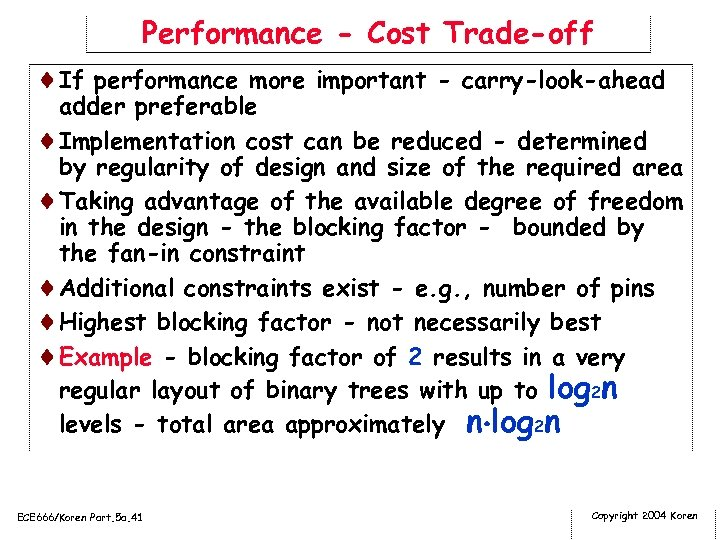Performance - Cost Trade-off ¨If performance more important - carry-look-ahead adder preferable ¨Implementation cost can be reduced - determined by regularity of design and size of the required area ¨Taking advantage of the available degree of freedom in the design - the blocking factor - bounded by the fan-in constraint ¨Additional constraints exist - e. g. , number of pins ¨Highest blocking factor - not necessarily best ¨Example - blocking factor of 2 results in a very regular layout of binary trees with up to log 2 n levels - total area approximately n log 2 n ECE 666/Koren Part. 5 a. 41 Copyright 2004 Koren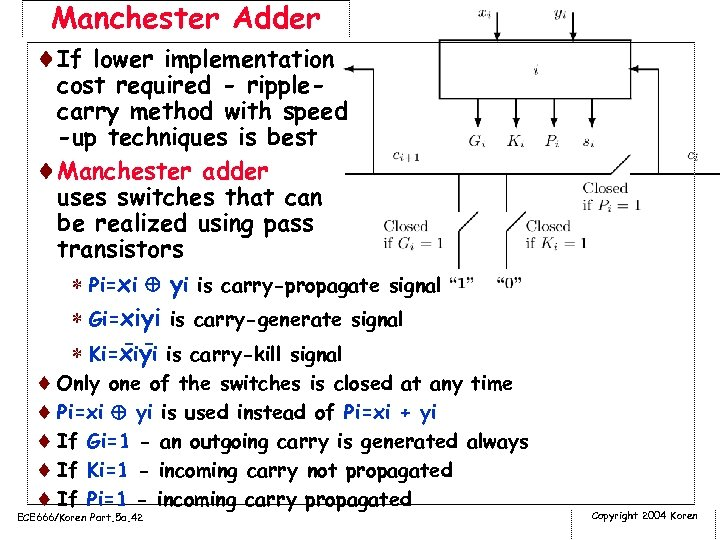Manchester Adder ¨If lower implementation cost required - ripplecarry method with speed -up techniques is best ¨Manchester adder uses switches that can be realized using pass transistors * Pi=xi yi is carry-propagate signal * Gi=xiyi is carry-generate signal -* Ki=xiyi is carry-kill signal ¨ Only one of the switches is closed at any time ¨ Pi=xi yi is used instead of Pi=xi + yi ¨ If Gi=1 - an outgoing carry is generated always ¨ If Ki=1 - incoming carry not propagated ¨ If Pi=1 - incoming carry propagated ECE 666/Koren Part. 5 a. 42 Copyright 2004 Koren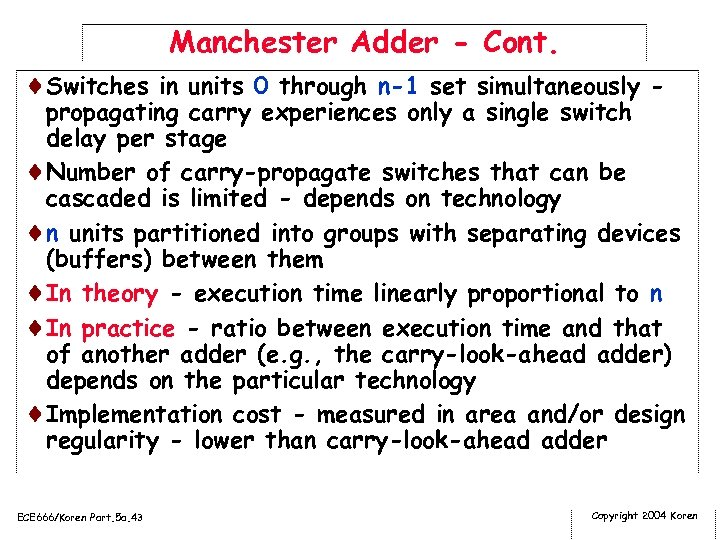Manchester Adder - Cont. ¨Switches in units 0 through n-1 set simultaneously - propagating carry experiences only a single switch delay per stage ¨Number of carry-propagate switches that can be cascaded is limited - depends on technology ¨n units partitioned into groups with separating devices (buffers) between them ¨In theory - execution time linearly proportional to n ¨In practice - ratio between execution time and that of another adder (e. g. , the carry-look-ahead adder) depends on the particular technology ¨Implementation cost - measured in area and/or design regularity - lower than carry-look-ahead adder ECE 666/Koren Part. 5 a. 43 Copyright 2004 Koren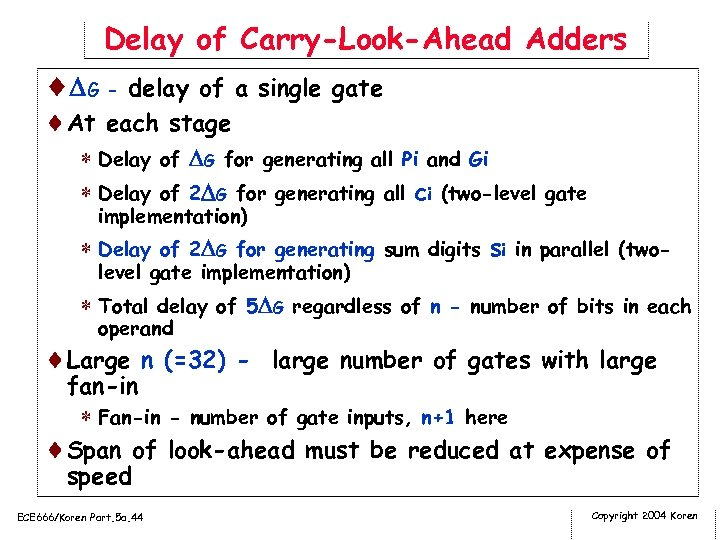Delay of Carry-Look-Ahead Adders ¨ G - delay of a single gate ¨At each stage * Delay of G for generating all Pi and Gi * Delay of 2 G for generating all ci (two-level gate implementation) * Delay of 2 G for generating sum digits si in parallel (twolevel gate implementation) * Total delay of 5 G regardless of n - number of bits in each operand ¨Large n (=32) - large number of gates with large fan-in * Fan-in - number of gate inputs, n+1 here ¨Span of look-ahead must be reduced at expense of speed ECE 666/Koren Part. 5 a. 44 Copyright 2004 Koren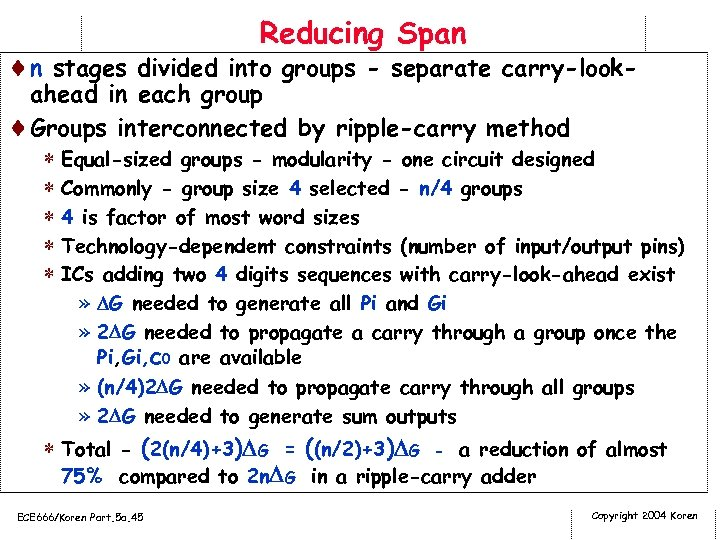Reducing Span ¨n stages divided into groups - separate carry-look- ahead in each group ¨Groups interconnected by ripple-carry method * Equal-sized groups - modularity - one circuit designed * Commonly - group size 4 selected - n/4 groups * 4 is factor of most word sizes * Technology-dependent constraints (number of input/output pins) * ICs adding two 4 digits sequences with carry-look-ahead exist » G needed to generate all Pi and Gi » 2 G needed to propagate a carry through a group once the Pi, Gi, c 0 are available » (n/4)2 G needed to propagate carry through all groups » 2 G needed to generate sum outputs * Total - (2(n/4)+3) G = ((n/2)+3) G - a reduction of almost 75% compared to 2 n G in a ripple-carry adder ECE 666/Koren Part. 5 a. 45 Copyright 2004 Koren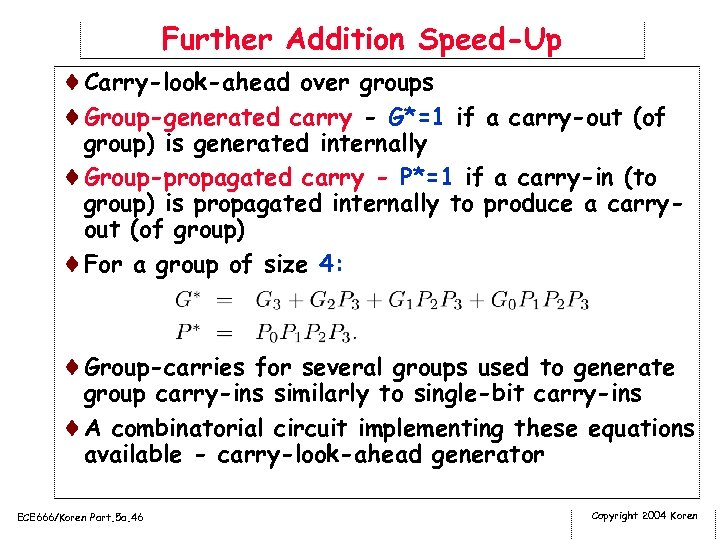Further Addition Speed-Up ¨Carry-look-ahead over groups ¨Group-generated carry - G*=1 if a carry-out (of group) is generated internally ¨Group-propagated carry - P*=1 if a carry-in (to group) is propagated internally to produce a carryout (of group) ¨For a group of size 4: ¨Group-carries for several groups used to generate group carry-ins similarly to single-bit carry-ins ¨A combinatorial circuit implementing these equations available - carry-look-ahead generator ECE 666/Koren Part. 5 a. 46 Copyright 2004 Koren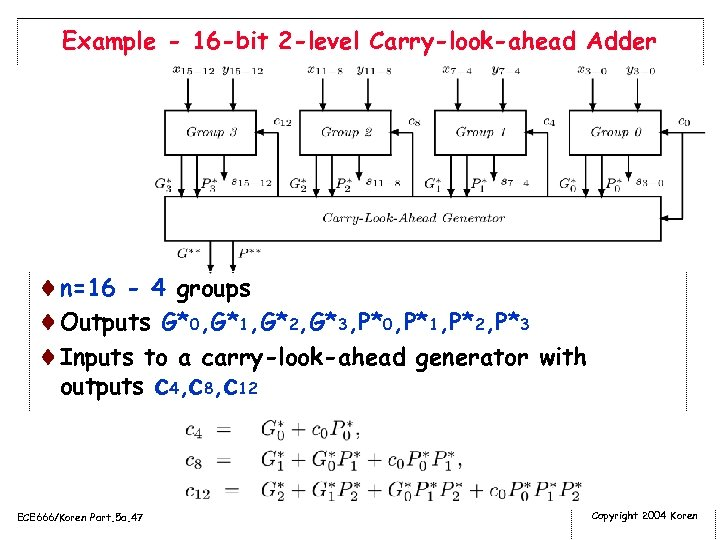Example - 16 -bit 2 -level Carry-look-ahead Adder ¨n=16 - 4 groups ¨Outputs G*0, G*1, G*2, G*3, P*0, P*1, P*2, P*3 ¨Inputs to a carry-look-ahead generator with outputs c 4, c 8, c 12 ECE 666/Koren Part. 5 a. 47 Copyright 2004 Koren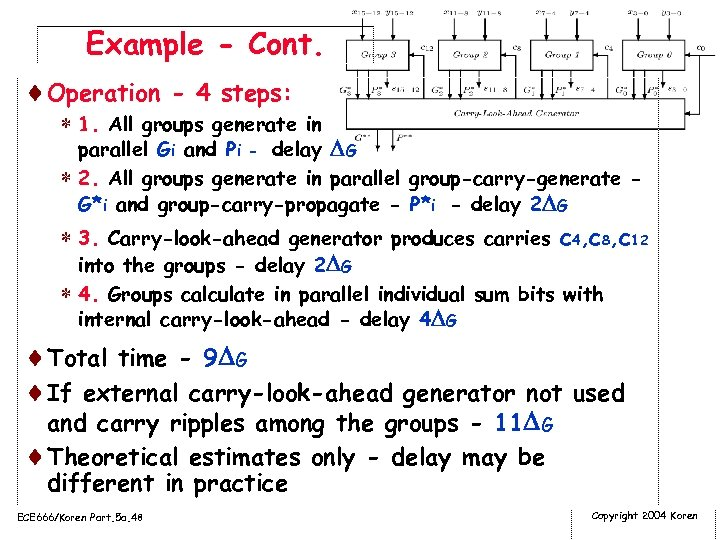Example - Cont. ¨Operation - 4 steps: * 1. All groups generate in parallel Gi and Pi - delay G * 2. All groups generate in parallel group-carry-generate G*i and group-carry-propagate - P*i - delay 2 G * 3. Carry-look-ahead generator produces carries c 4, c 8, c 12 into the groups - delay 2 G * 4. Groups calculate in parallel individual sum bits with internal carry-look-ahead - delay 4 G ¨Total time - 9 G ¨If external carry-look-ahead generator not used and carry ripples among the groups - 11 G ¨Theoretical estimates only - delay may be different in practice ECE 666/Koren Part. 5 a. 48 Copyright 2004 Koren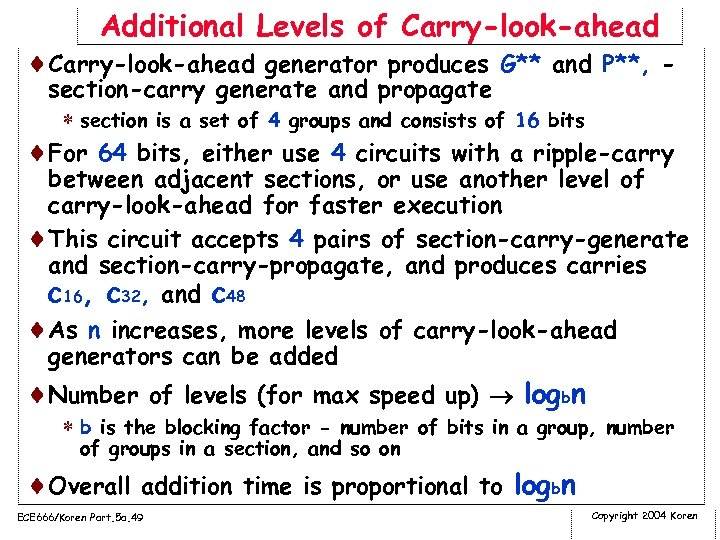Additional Levels of Carry-look-ahead ¨Carry-look-ahead generator produces G** and P**, - section-carry generate and propagate * section is a set of 4 groups and consists of 16 bits ¨For 64 bits, either use 4 circuits with a ripple-carry between adjacent sections, or use another level of carry-look-ahead for faster execution ¨This circuit accepts 4 pairs of section-carry-generate and section-carry-propagate, and produces carries c 16, c 32, and c 48 ¨As n increases, more levels of carry-look-ahead generators can be added ¨Number of levels (for max speed up) logbn * b is the blocking factor - number of bits in a group, number of groups in a section, and so on ¨Overall addition time is proportional to logbn ECE 666/Koren Part. 5 a. 49 Copyright 2004 Koren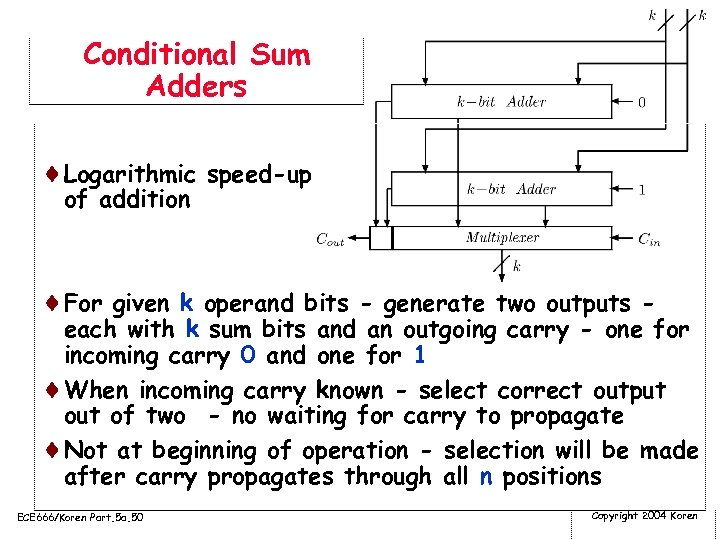Conditional Sum Adders ¨Logarithmic speed-up of addition ¨For given k operand bits - generate two outputs - each with k sum bits and an outgoing carry - one for incoming carry 0 and one for 1 ¨When incoming carry known - select correct output of two - no waiting for carry to propagate ¨Not at beginning of operation - selection will be made after carry propagates through all n positions ECE 666/Koren Part. 5 a. 50 Copyright 2004 Koren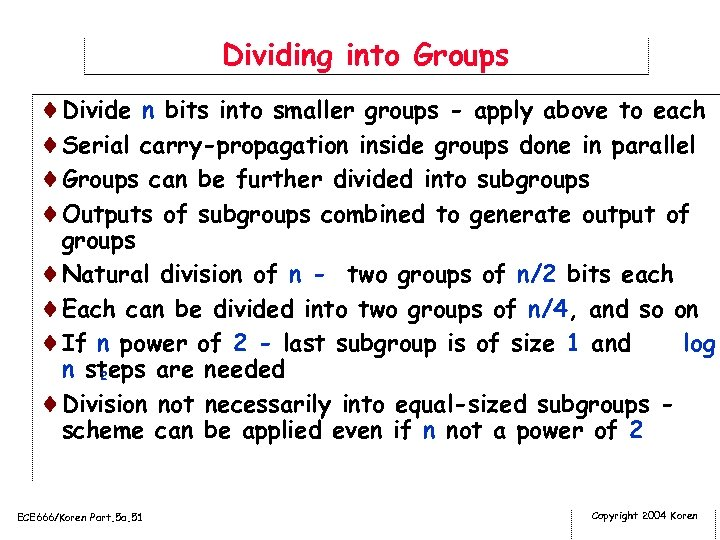Dividing into Groups ¨Divide n bits into smaller groups - apply above to each ¨Serial carry-propagation inside groups done in parallel ¨Groups can be further divided into subgroups ¨Outputs of subgroups combined to generate output of groups ¨Natural division of n - two groups of n/2 bits each ¨Each can be divided into two groups of n/4, and so on ¨If n power of 2 - last subgroup is of size 1 and log n steps are needed 2 ¨Division not necessarily into equal-sized subgroups scheme can be applied even if n not a power of 2 ECE 666/Koren Part. 5 a. 51 Copyright 2004 Koren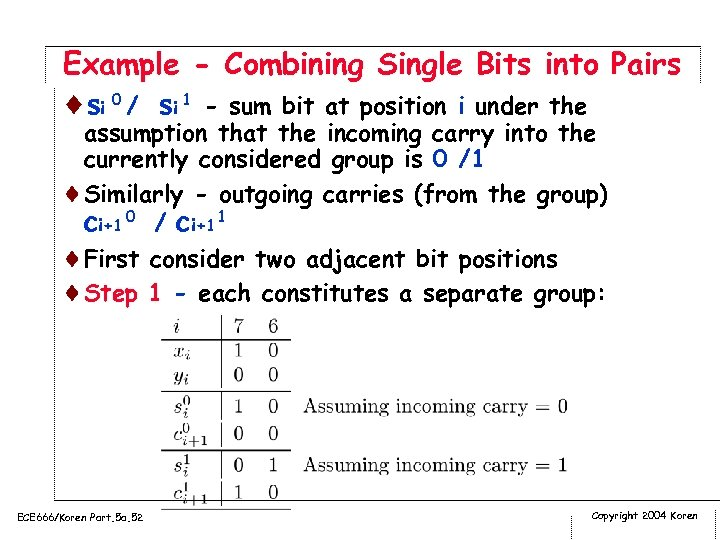Example - Combining Single Bits into Pairs ¨ si 0 / si 1 - sum bit at position i under the assumption that the incoming carry into the currently considered group is 0 /1 ¨Similarly - outgoing carries (from the group) ci+1 0 / ci+1 1 ¨First consider two adjacent bit positions ¨Step 1 - each constitutes a separate group: ECE 666/Koren Part. 5 a. 52 Copyright 2004 Koren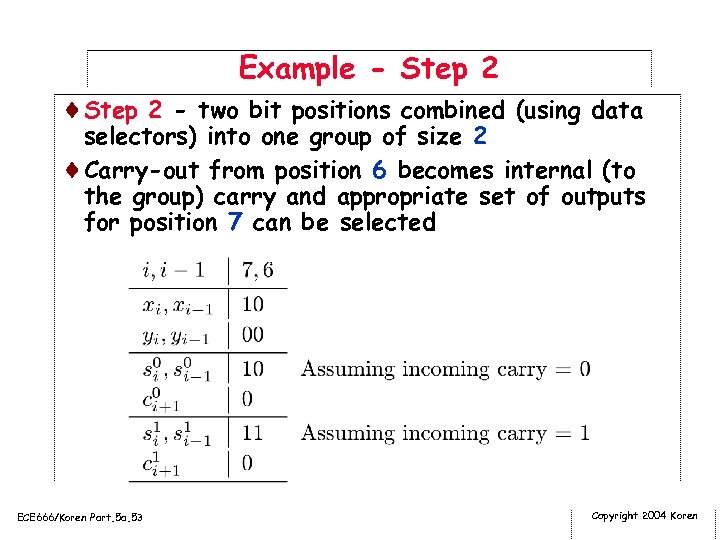Example - Step 2 ¨Step 2 - two bit positions combined (using data selectors) into one group of size 2 ¨Carry-out from position 6 becomes internal (to the group) carry and appropriate set of outputs for position 7 can be selected ECE 666/Koren Part. 5 a. 53 Copyright 2004 Koren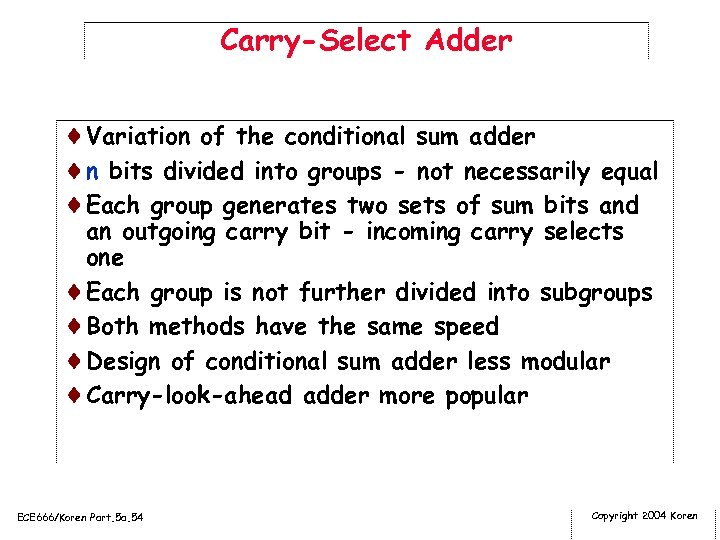Carry-Select Adder ¨Variation of the conditional sum adder ¨n bits divided into groups - not necessarily equal ¨Each group generates two sets of sum bits and an outgoing carry bit - incoming carry selects one ¨Each group is not further divided into subgroups ¨Both methods have the same speed ¨Design of conditional sum adder less modular ¨Carry-look-ahead adder more popular ECE 666/Koren Part. 5 a. 54 Copyright 2004 Koren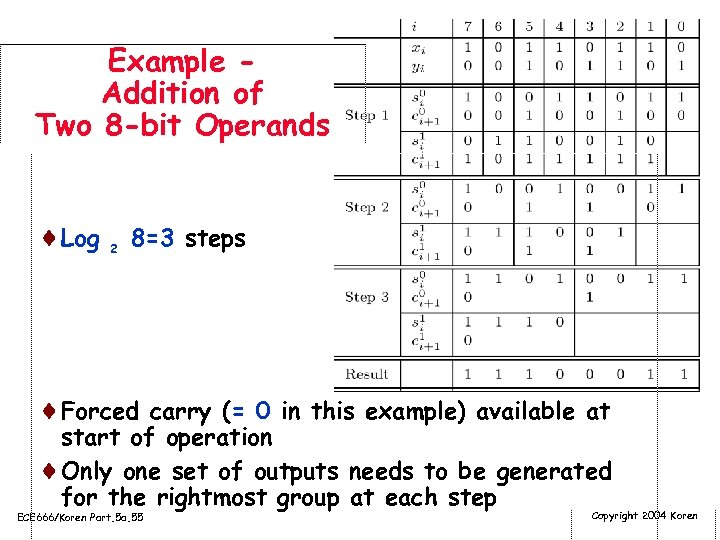Example Addition of Two 8 -bit Operands ¨Log 2 8=3 steps ¨Forced carry (= 0 in this example) available at start of operation ¨Only one set of outputs needs to be generated for the rightmost group at each step ECE 666/Koren Part. 5 a. 55 Copyright 2004 Koren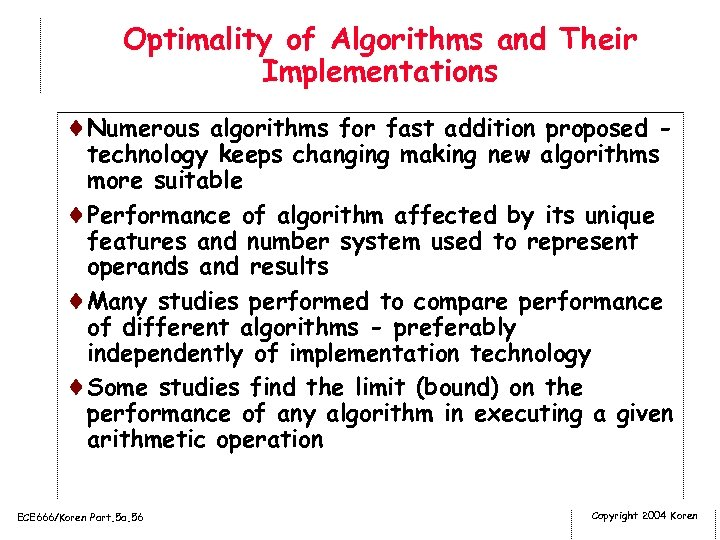Optimality of Algorithms and Their Implementations ¨Numerous algorithms for fast addition proposed - technology keeps changing making new algorithms more suitable ¨Performance of algorithm affected by its unique features and number system used to represent operands and results ¨Many studies performed to compare performance of different algorithms - preferably independently of implementation technology ¨Some studies find the limit (bound) on the performance of any algorithm in executing a given arithmetic operation ECE 666/Koren Part. 5 a. 56 Copyright 2004 Koren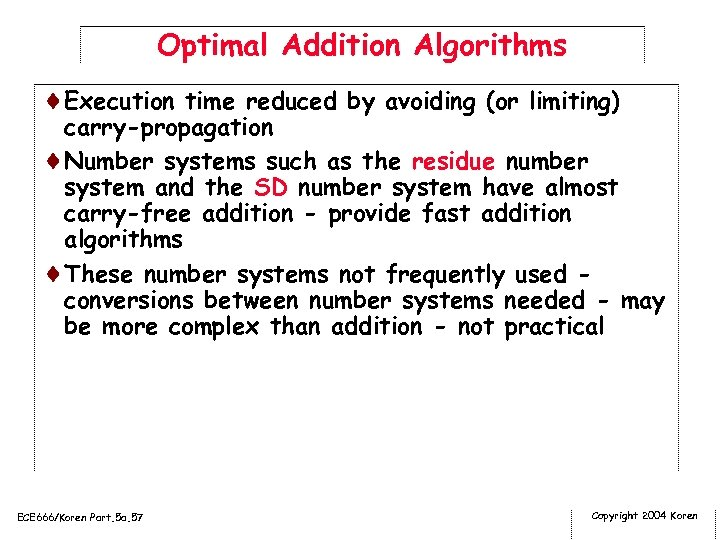Optimal Addition Algorithms ¨Execution time reduced by avoiding (or limiting) carry-propagation ¨Number systems such as the residue number system and the SD number system have almost carry-free addition - provide fast addition algorithms ¨These number systems not frequently used conversions between number systems needed - may be more complex than addition - not practical ECE 666/Koren Part. 5 a. 57 Copyright 2004 Koren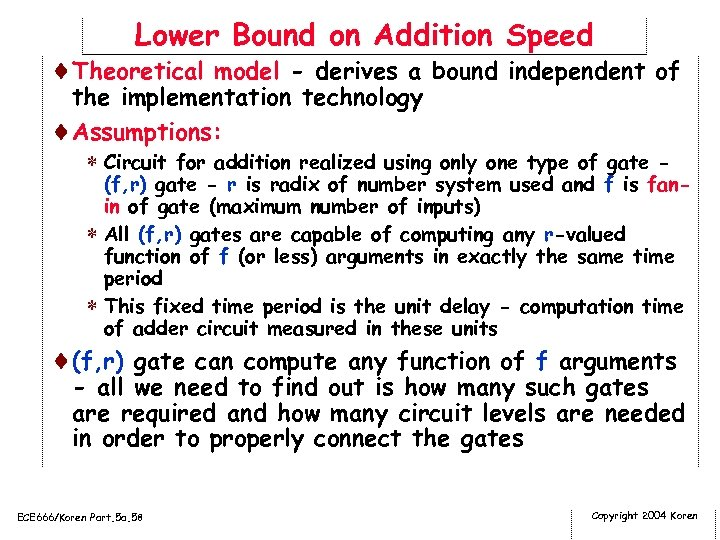Lower Bound on Addition Speed ¨Theoretical model - derives a bound independent of the implementation technology ¨Assumptions: * Circuit for addition realized using only one type of gate - (f, r) gate - r is radix of number system used and f is fanin of gate (maximum number of inputs) * All (f, r) gates are capable of computing any r-valued function of f (or less) arguments in exactly the same time period * This fixed time period is the unit delay - computation time of adder circuit measured in these units ¨(f, r) gate can compute any function of f arguments - all we need to find out is how many such gates are required and how many circuit levels are needed in order to properly connect the gates ECE 666/Koren Part. 5 a. 58 Copyright 2004 Koren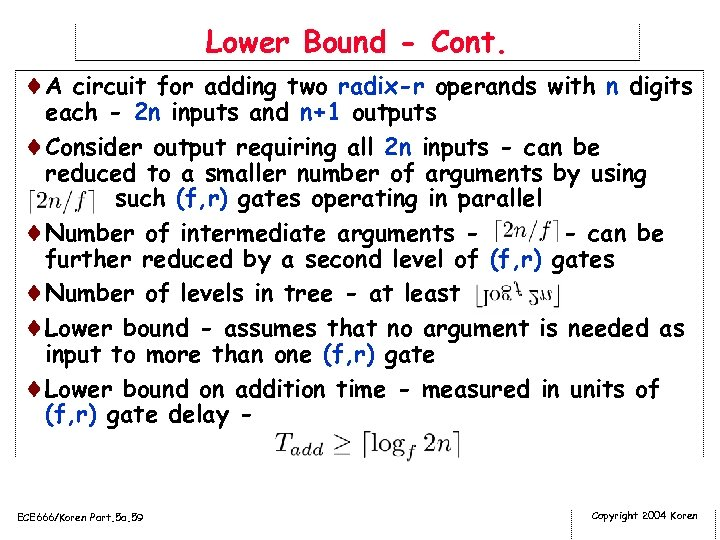Lower Bound - Cont. ¨A circuit for adding two radix-r operands with n digits each - 2 n inputs and n+1 outputs ¨Consider output requiring all 2 n inputs - can be reduced to a smaller number of arguments by using such (f, r) gates operating in parallel ¨Number of intermediate arguments - can be further reduced by a second level of (f, r) gates ¨Number of levels in tree - at least ¨Lower bound - assumes that no argument is needed as input to more than one (f, r) gate ¨Lower bound on addition time - measured in units of (f, r) gate delay - ECE 666/Koren Part. 5 a. 59 Copyright 2004 Koren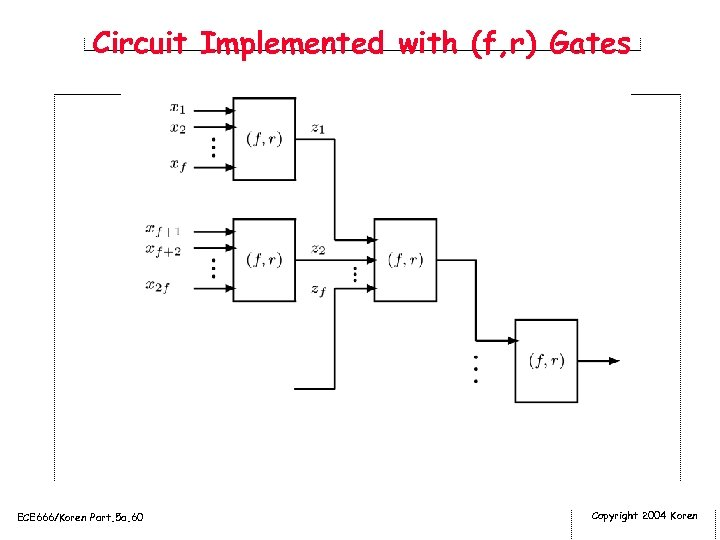Circuit Implemented with (f, r) Gates ECE 666/Koren Part. 5 a. 60 Copyright 2004 Koren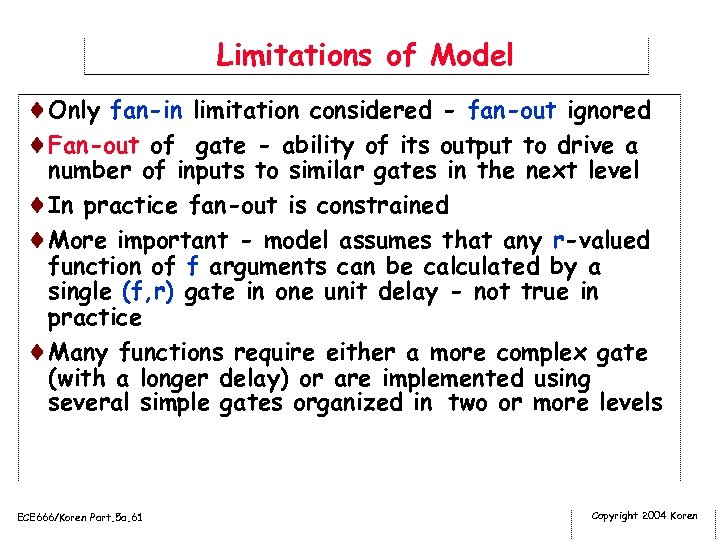Limitations of Model ¨Only fan-in limitation considered - fan-out ignored ¨Fan-out of gate - ability of its output to drive a number of inputs to similar gates in the next level ¨In practice fan-out is constrained ¨More important - model assumes that any r-valued function of f arguments can be calculated by a single (f, r) gate in one unit delay - not true in practice ¨Many functions require either a more complex gate (with a longer delay) or are implemented using several simple gates organized in two or more levels ECE 666/Koren Part. 5 a. 61 Copyright 2004 Koren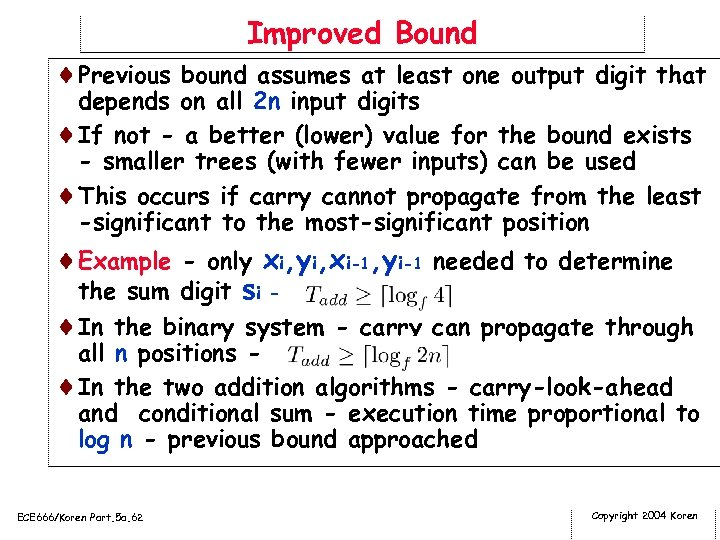Improved Bound ¨Previous bound assumes at least one output digit that depends on all 2 n input digits ¨If not - a better (lower) value for the bound exists - smaller trees (with fewer inputs) can be used ¨This occurs if carry cannot propagate from the least -significant to the most-significant position ¨Example - only xi, yi, xi-1, yi-1 needed to determine the sum digit si ¨In the binary system - carry can propagate through all n positions ¨In the two addition algorithms - carry-look-ahead and conditional sum - execution time proportional to log n - previous bound approached ECE 666/Koren Part. 5 a. 62 Copyright 2004 Koren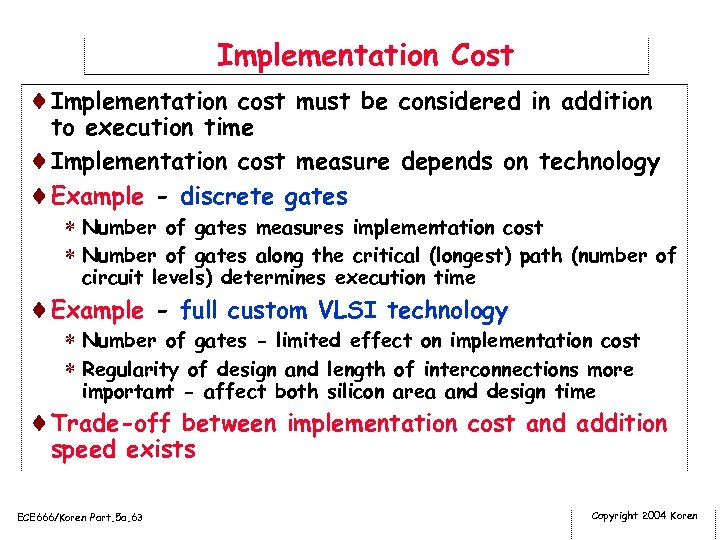Implementation Cost ¨Implementation cost must be considered in addition to execution time ¨Implementation cost measure depends on technology ¨Example - discrete gates * Number of gates measures implementation cost * Number of gates along the critical (longest) path (number of circuit levels) determines execution time ¨Example - full custom VLSI technology * Number of gates - limited effect on implementation cost * Regularity of design and length of interconnections more important - affect both silicon area and design time ¨Trade-off between implementation cost and addition speed exists ECE 666/Koren Part. 5 a. 63 Copyright 2004 Koren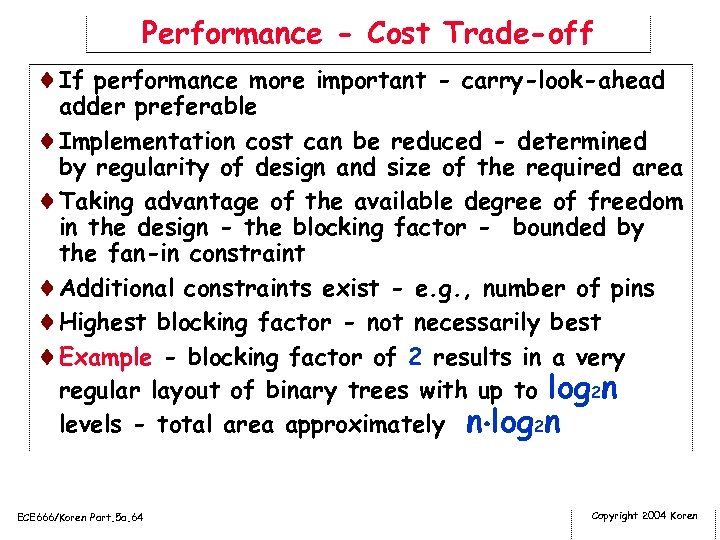Performance - Cost Trade-off ¨If performance more important - carry-look-ahead adder preferable ¨Implementation cost can be reduced - determined by regularity of design and size of the required area ¨Taking advantage of the available degree of freedom in the design - the blocking factor - bounded by the fan-in constraint ¨Additional constraints exist - e. g. , number of pins ¨Highest blocking factor - not necessarily best ¨Example - blocking factor of 2 results in a very regular layout of binary trees with up to log 2 n levels - total area approximately n log 2 n ECE 666/Koren Part. 5 a. 64 Copyright 2004 Koren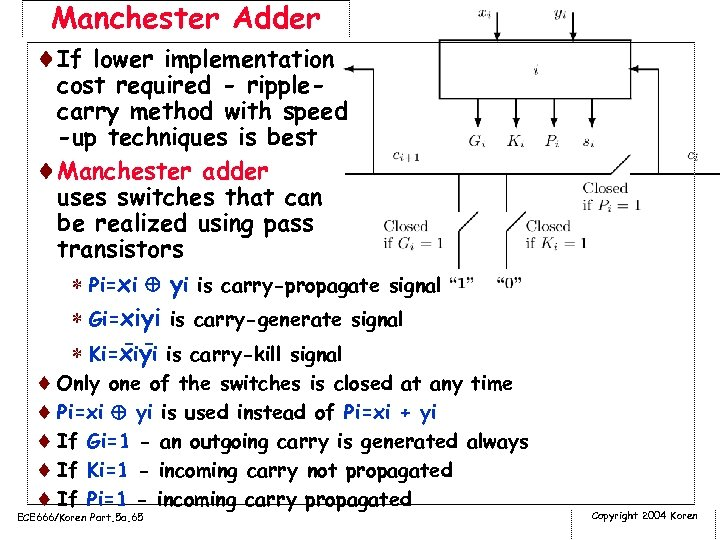Manchester Adder ¨If lower implementation cost required - ripplecarry method with speed -up techniques is best ¨Manchester adder uses switches that can be realized using pass transistors * Pi=xi yi is carry-propagate signal * Gi=xiyi is carry-generate signal -* Ki=xiyi is carry-kill signal ¨ Only one of the switches is closed at any time ¨ Pi=xi yi is used instead of Pi=xi + yi ¨ If Gi=1 - an outgoing carry is generated always ¨ If Ki=1 - incoming carry not propagated ¨ If Pi=1 - incoming carry propagated ECE 666/Koren Part. 5 a. 65 Copyright 2004 Koren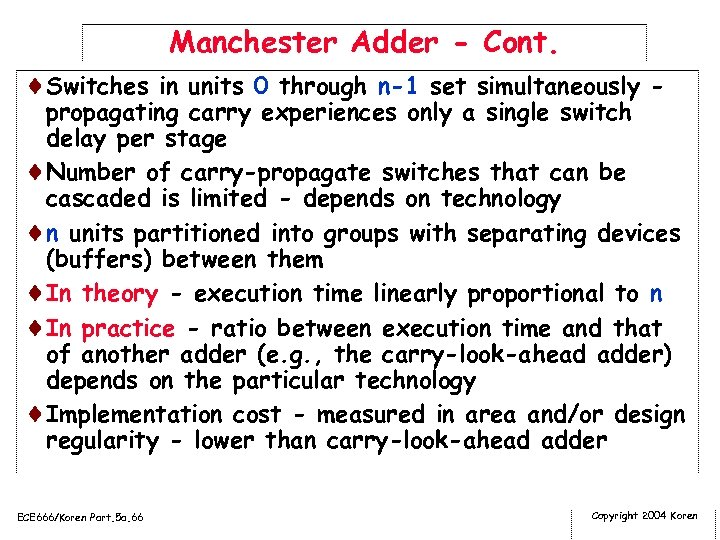Manchester Adder - Cont. ¨Switches in units 0 through n-1 set simultaneously - propagating carry experiences only a single switch delay per stage ¨Number of carry-propagate switches that can be cascaded is limited - depends on technology ¨n units partitioned into groups with separating devices (buffers) between them ¨In theory - execution time linearly proportional to n ¨In practice - ratio between execution time and that of another adder (e. g. , the carry-look-ahead adder) depends on the particular technology ¨Implementation cost - measured in area and/or design regularity - lower than carry-look-ahead adder ECE 666/Koren Part. 5 a. 66 Copyright 2004 Koren